# Selina Solutions Concise Maths Class 7 Chapter 11: Fundamental Concepts (Including Fundamental Operations)

Selina Solutions Concise Maths Class 7 Chapter 11 Fundamental Concepts (Including Fundamental Operations) help students get a clear idea of operations and methods to be followed in solving problems effortlessly. The solutions contain explanations of basic concepts in an understandable language. Shortcut tricks and important formulas are covered in a stepwise manner for better conceptual knowledge among students. Selina Solutions Concise Maths Class 7 Chapter 11 Fundamental Concepts (Including Fundamental Operations) PDF can be downloaded from the links provided below.

Chapter 11 explains the elementary treatment and operations on various terms, as per the latest syllabus of the ICSE board. The main aim of preparing chapter-wise solutions is to provide a better hold on the subject among students.

## Selina Solutions Concise Maths Class 7 Chapter 11: Fundamental Concepts (Including Fundamental Operations) Download PDF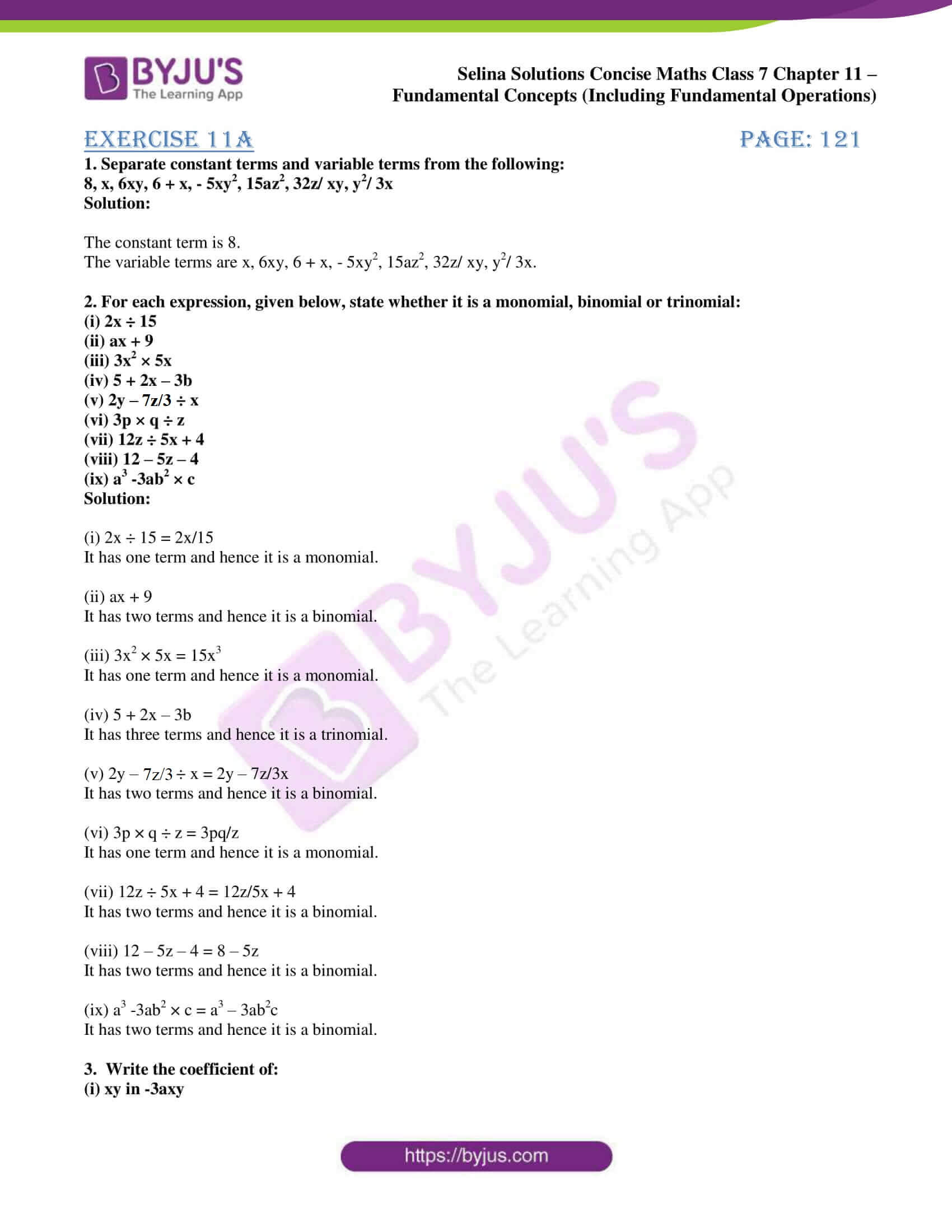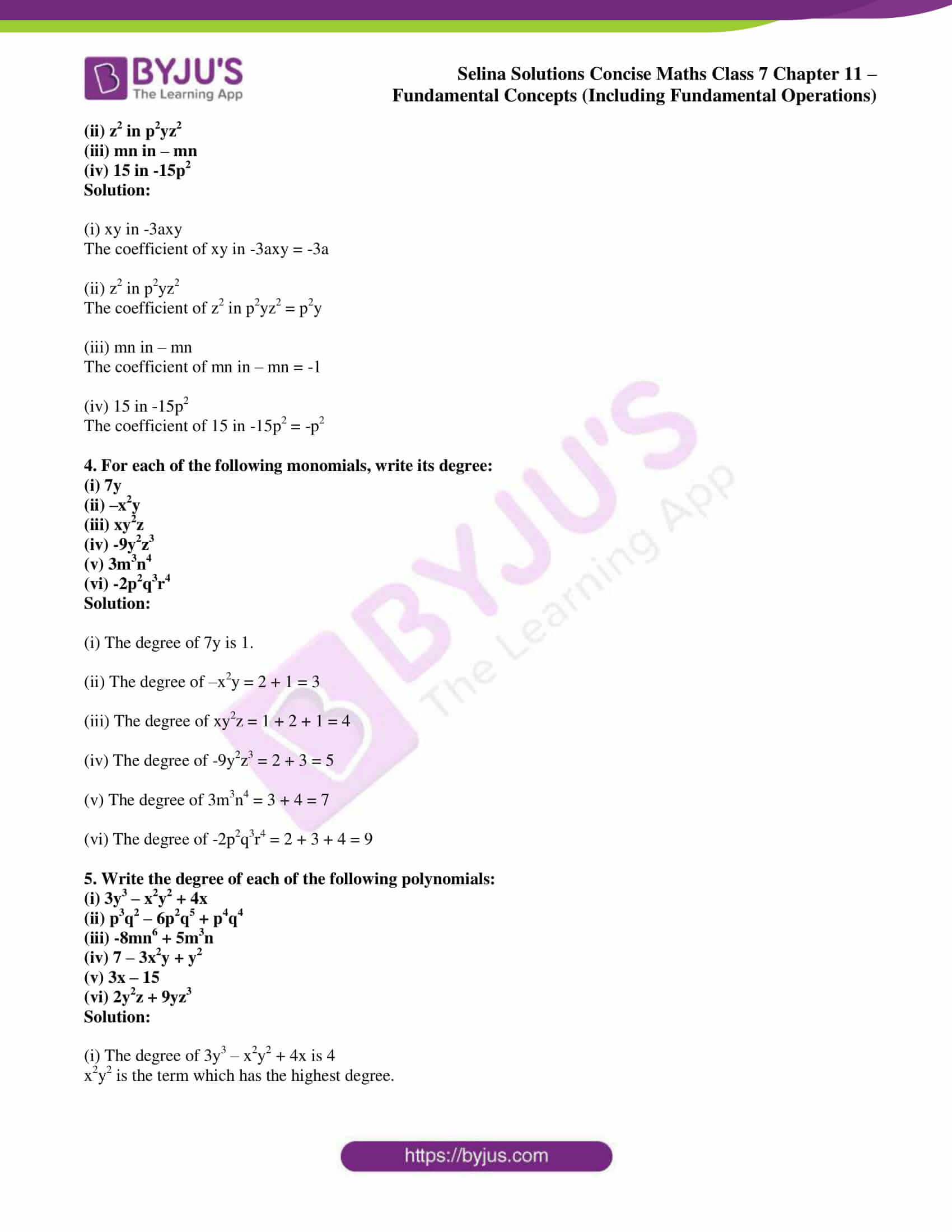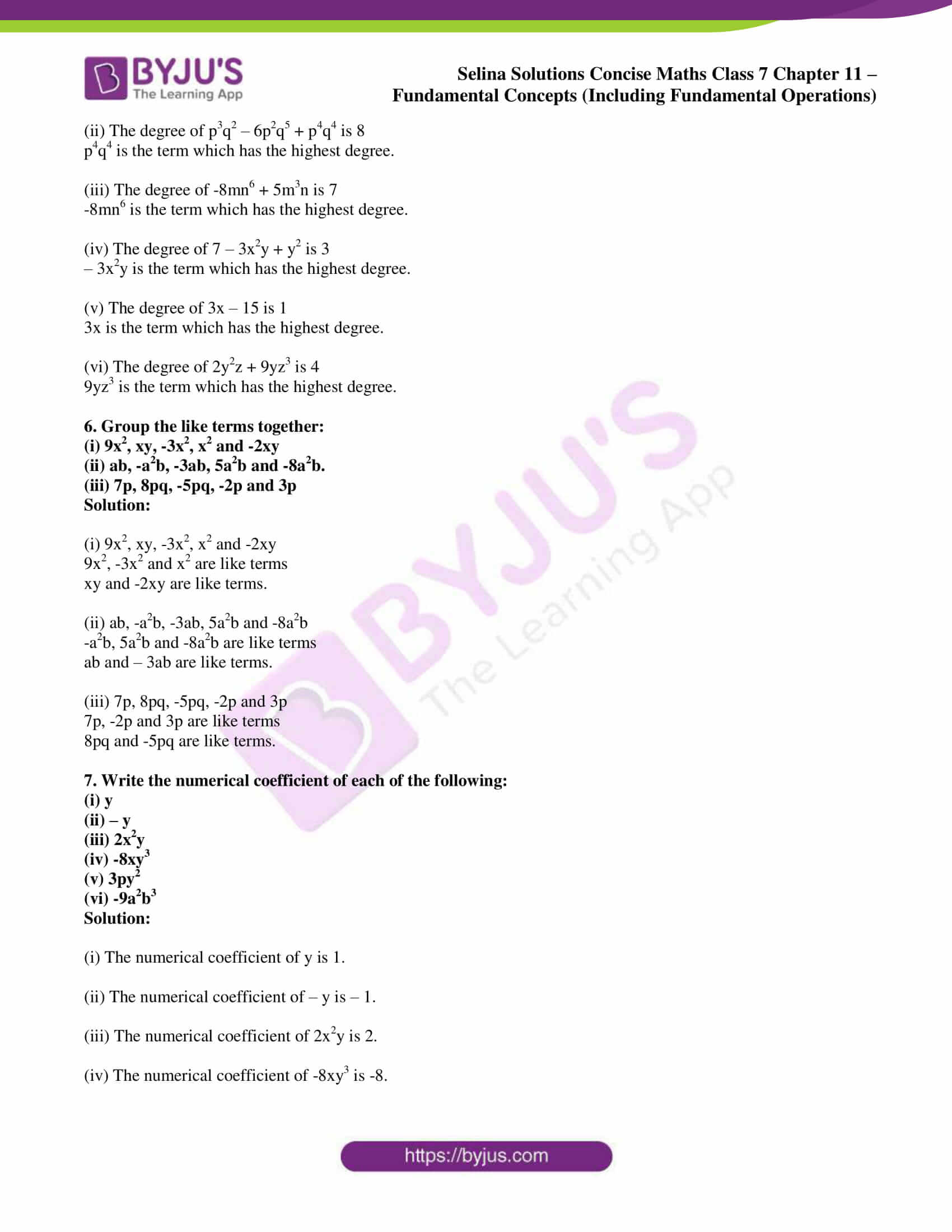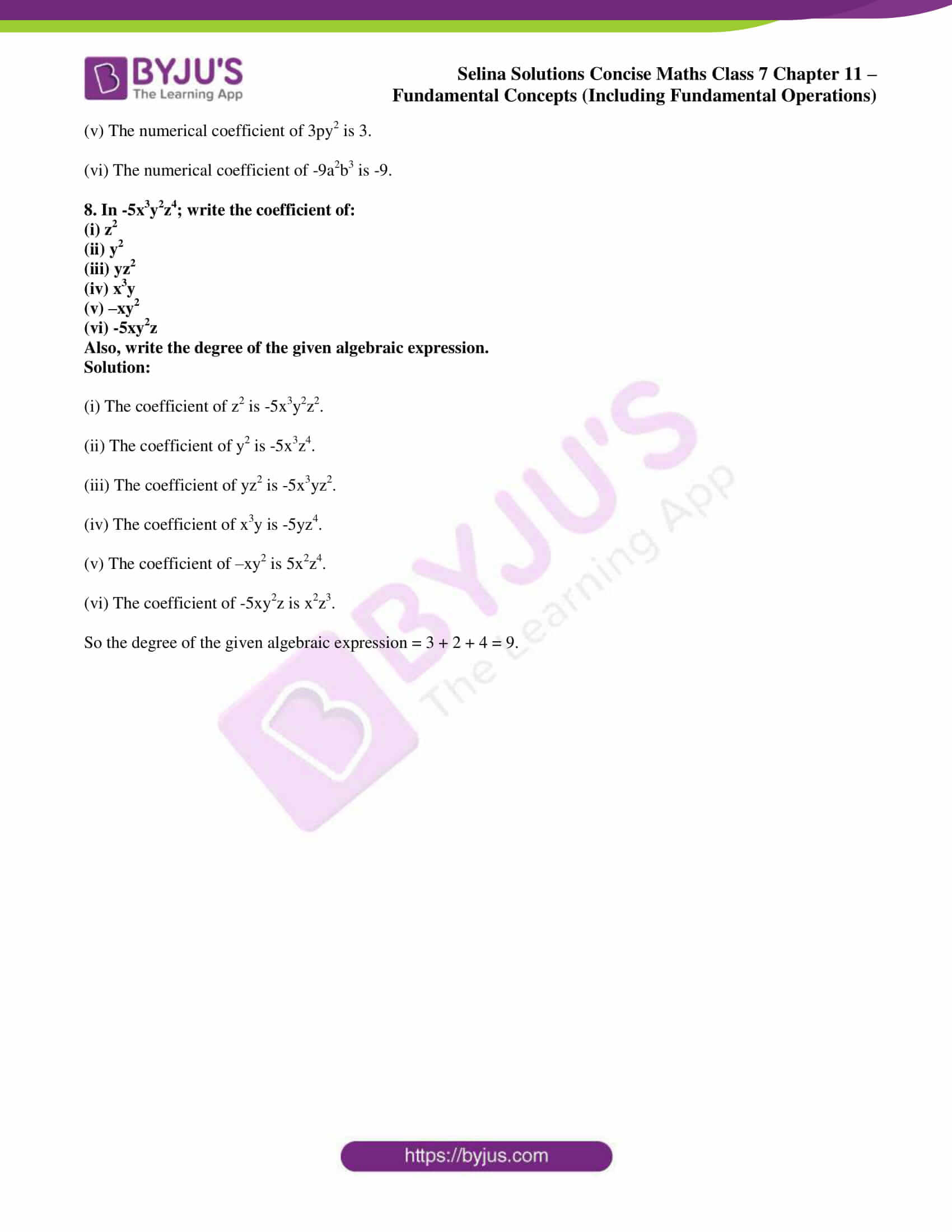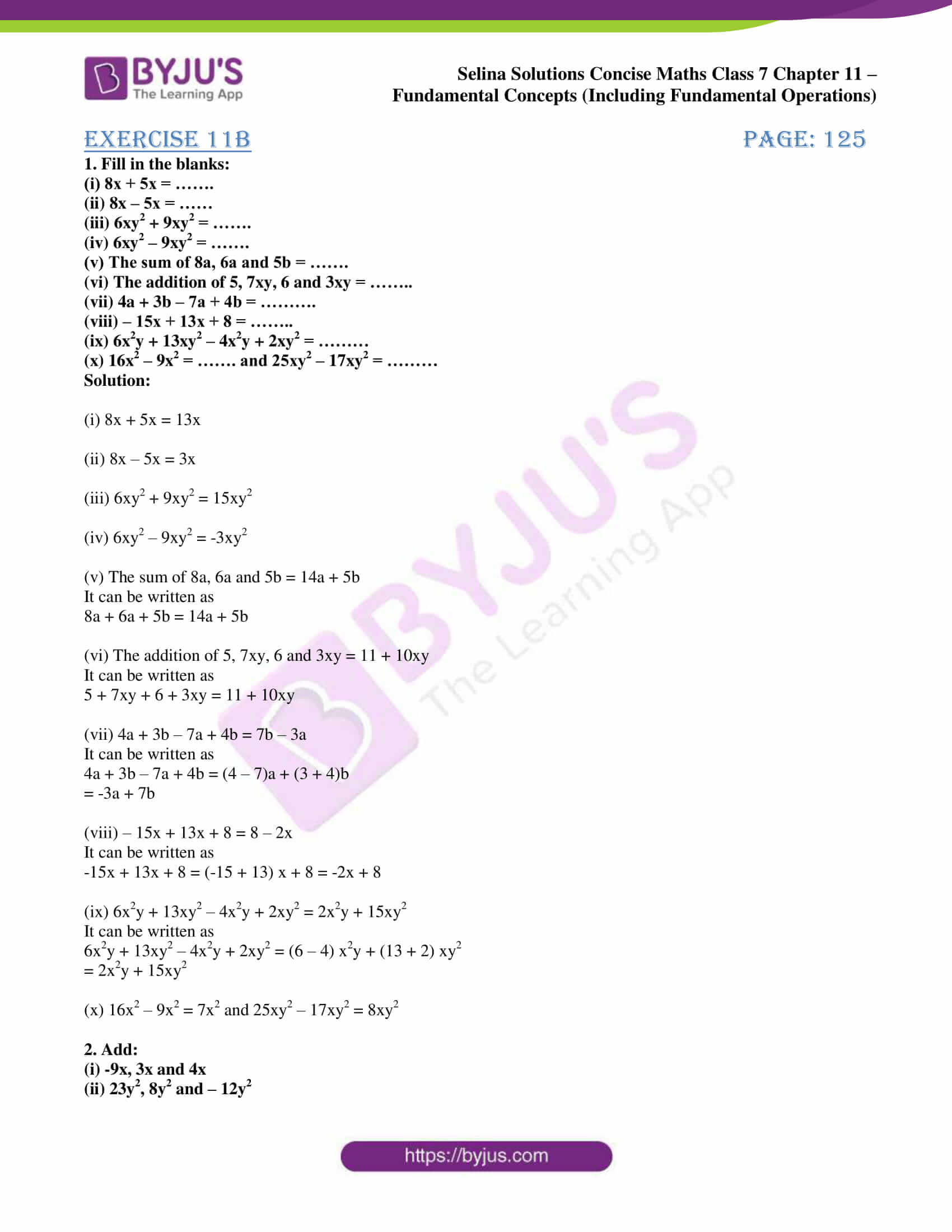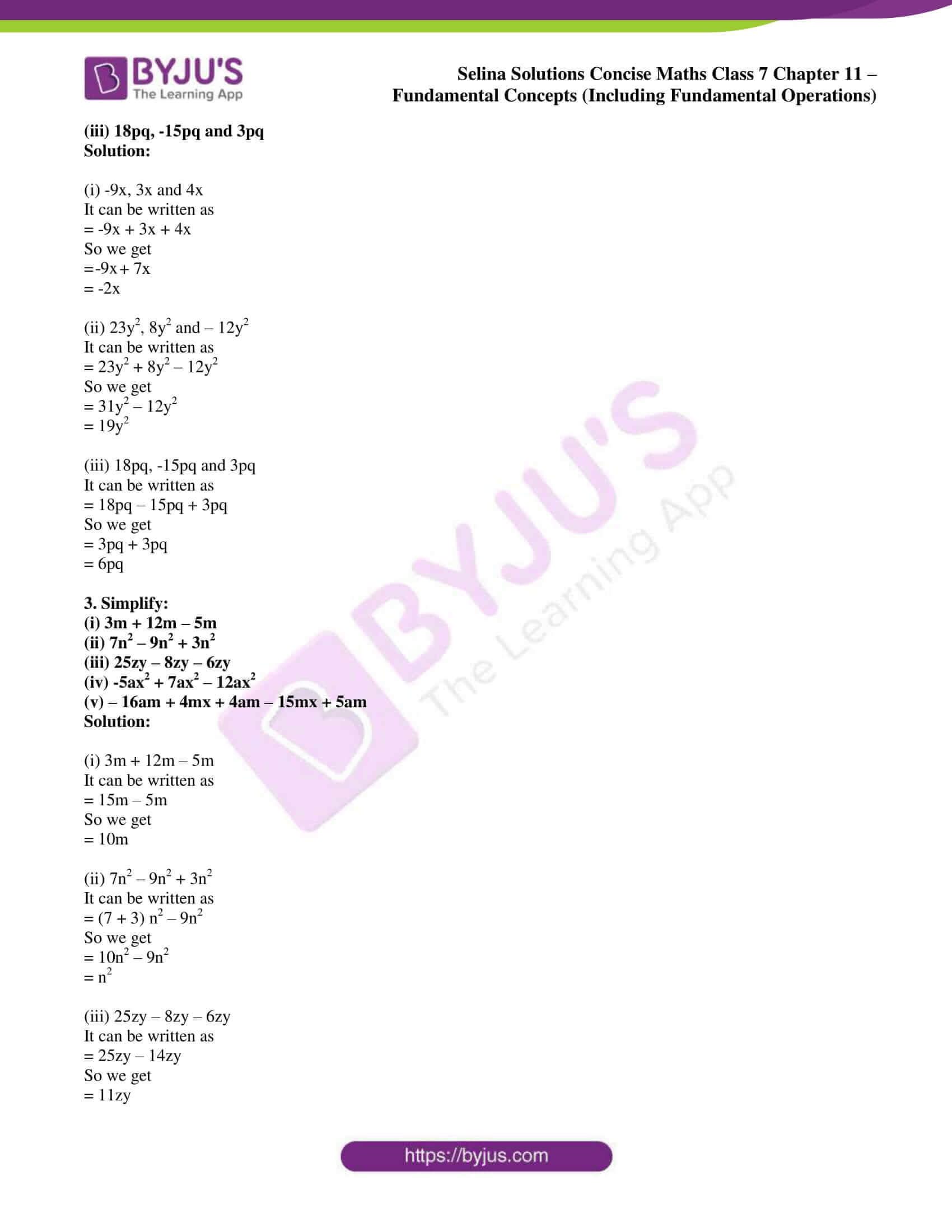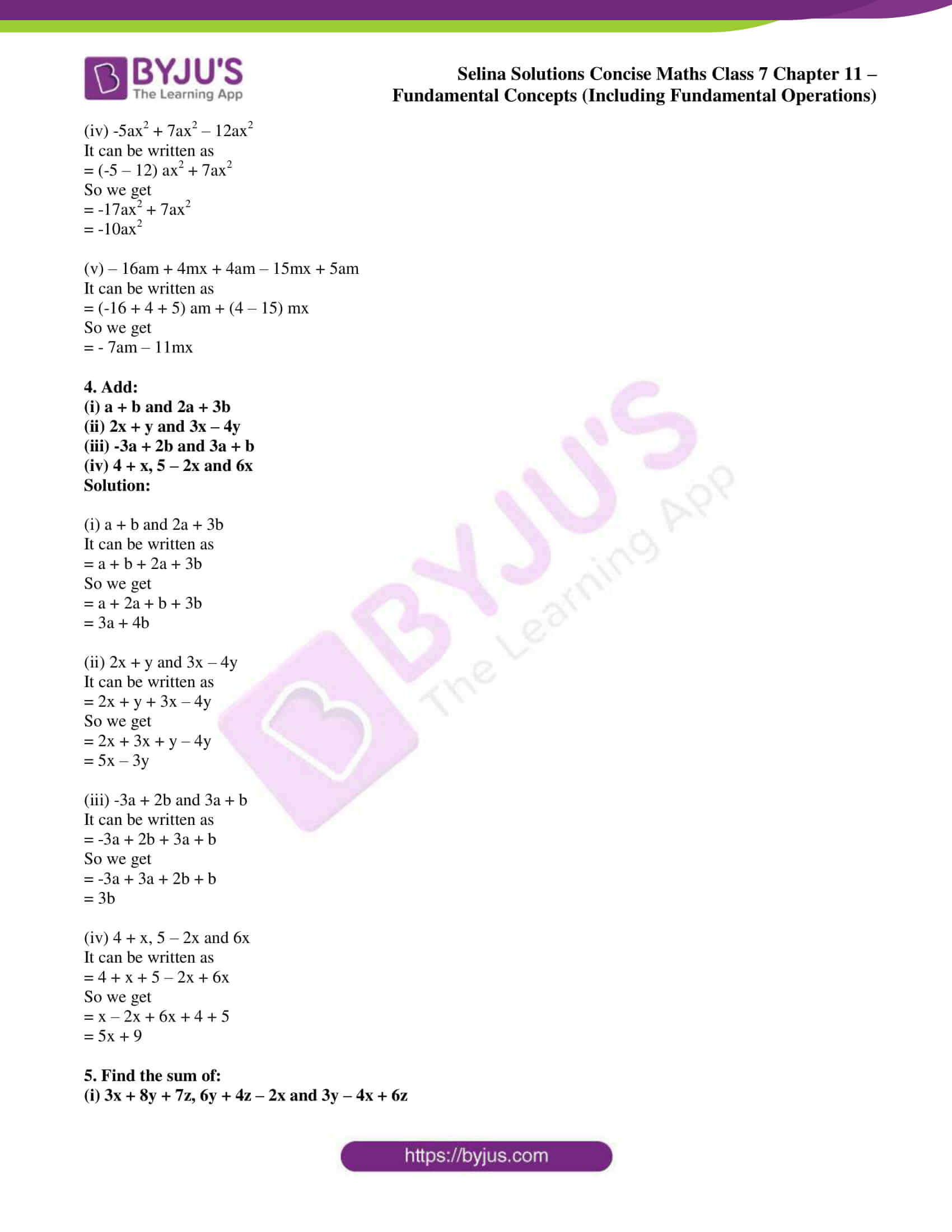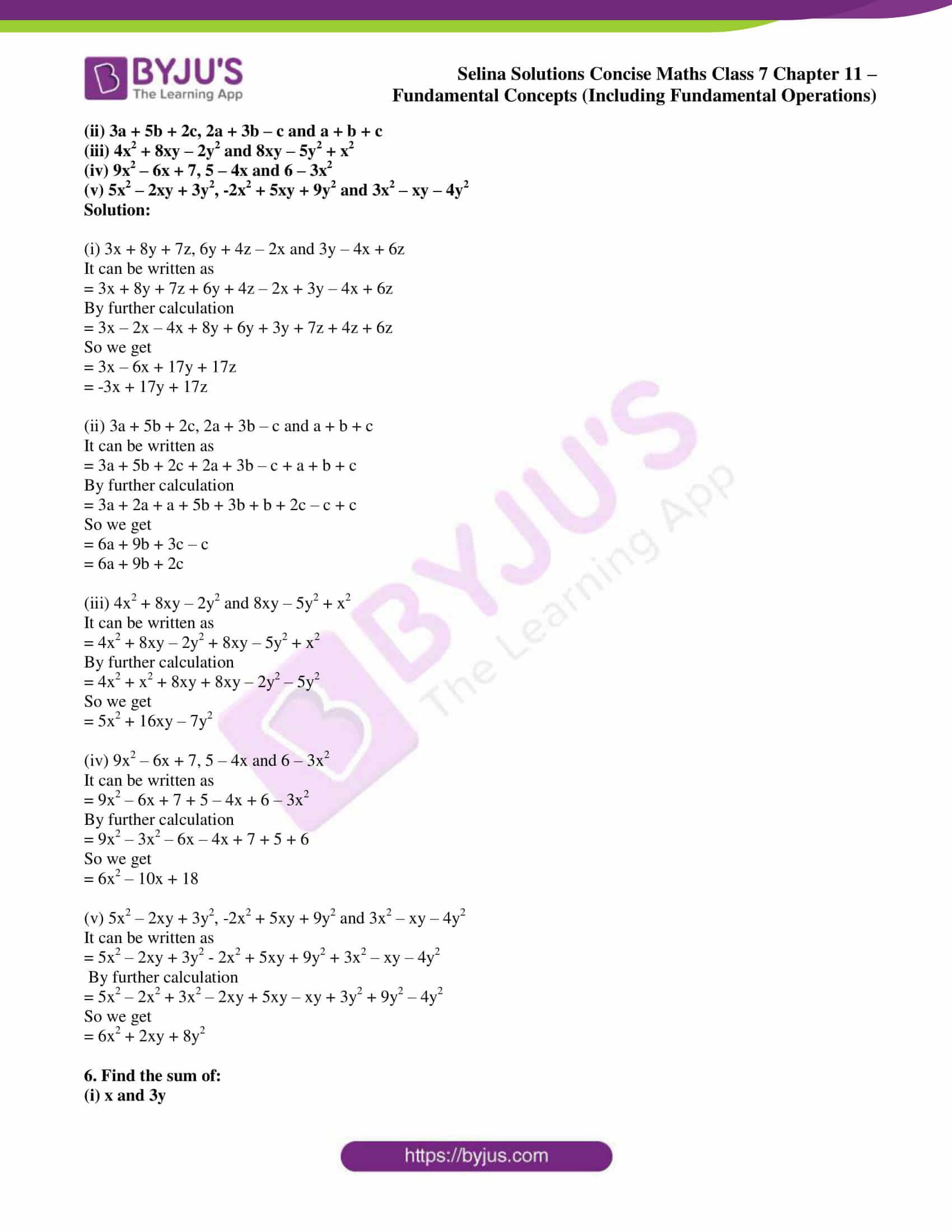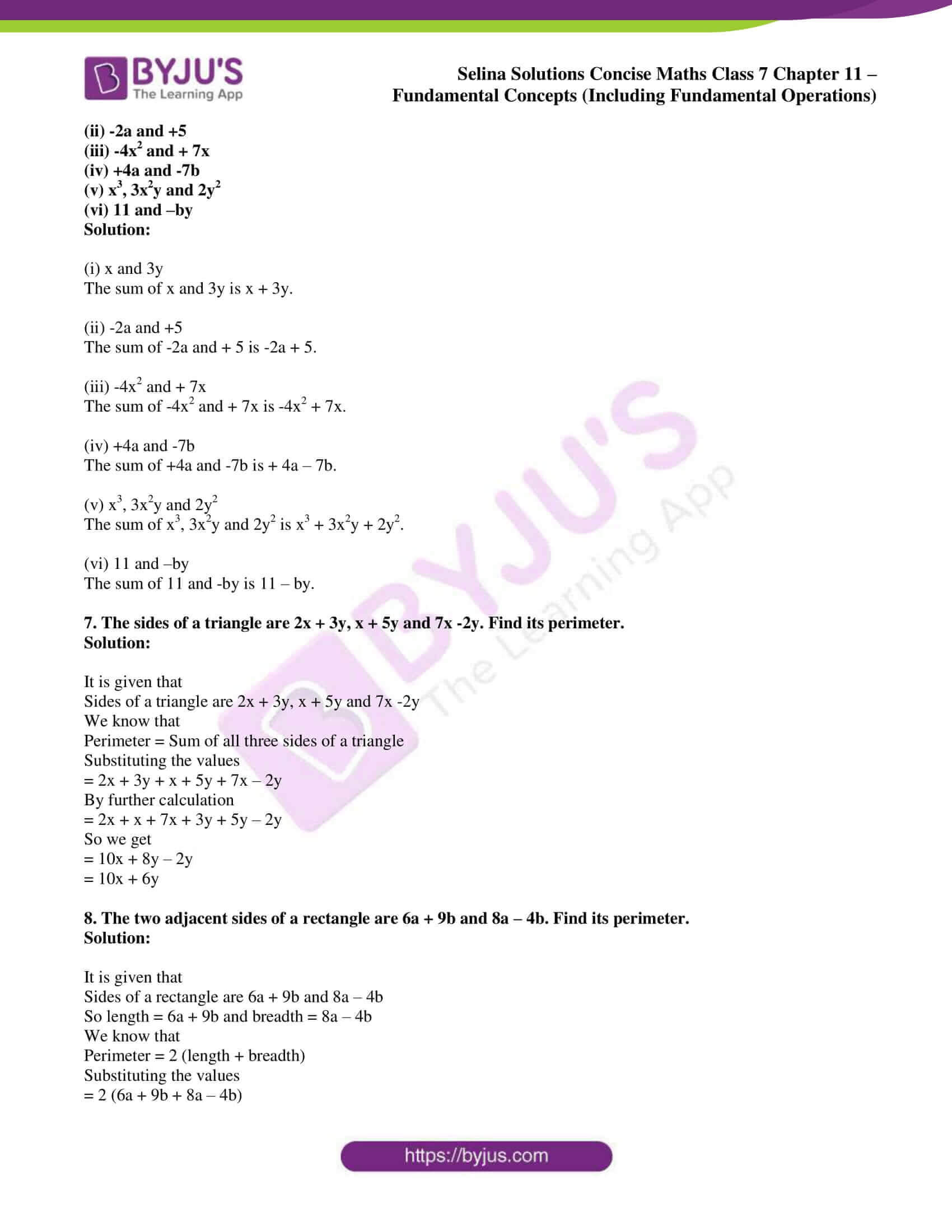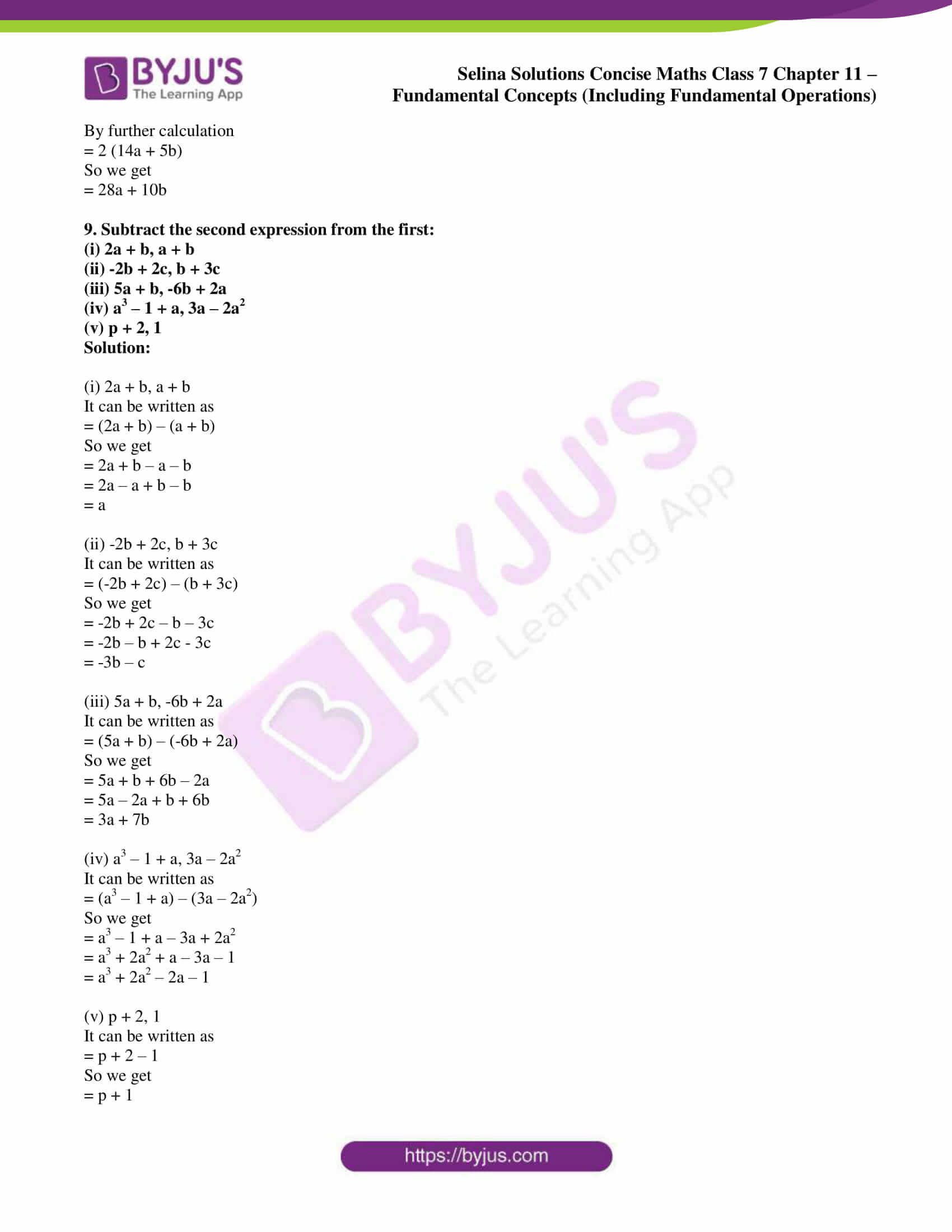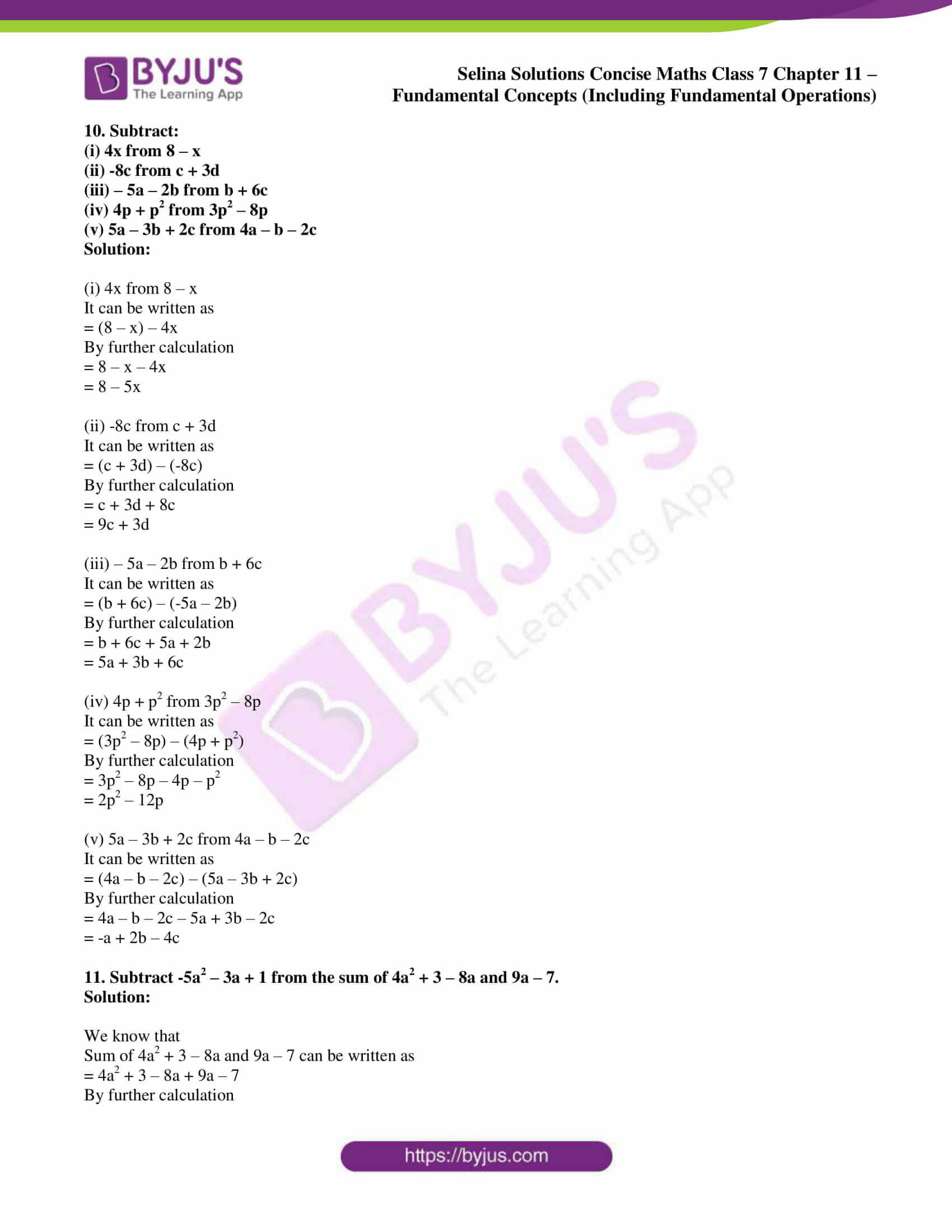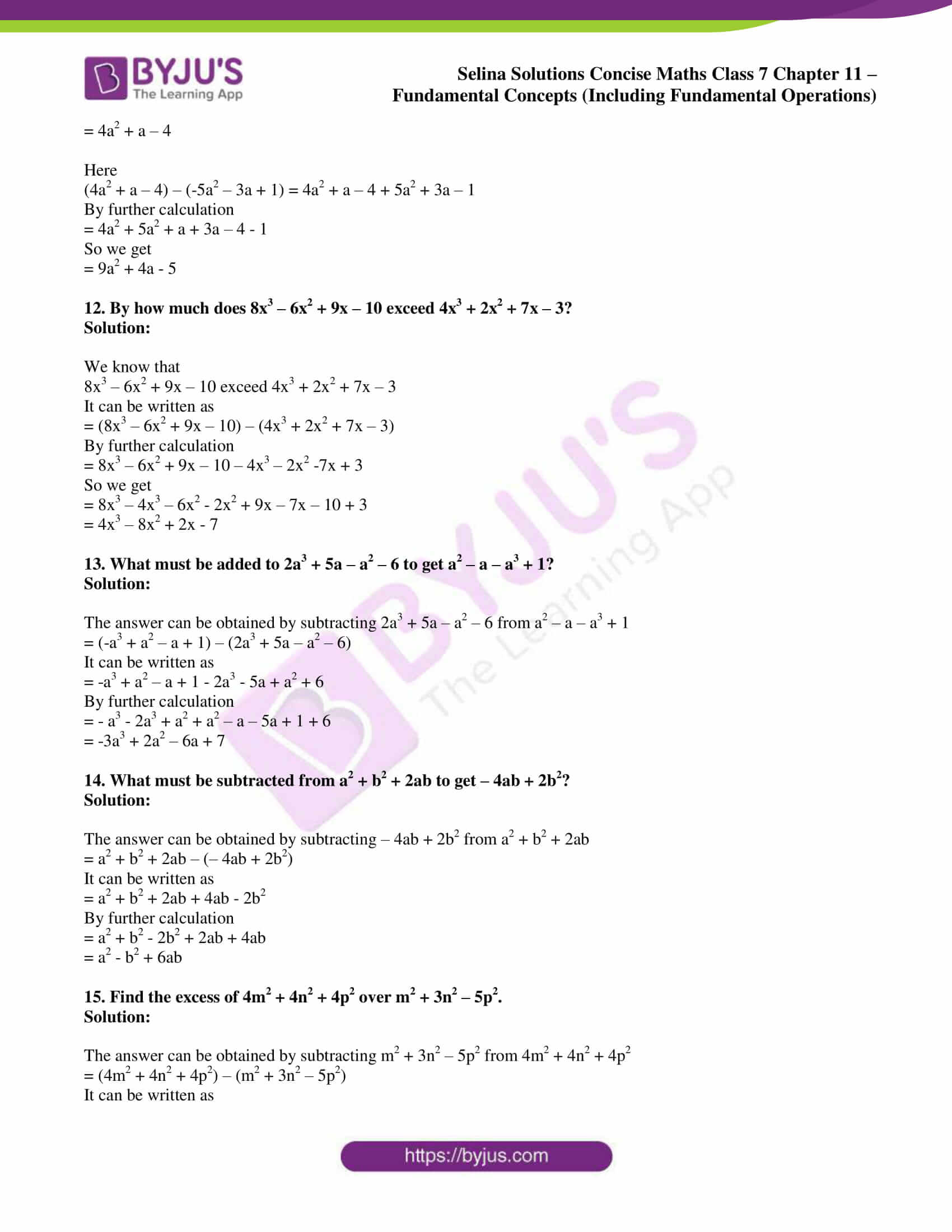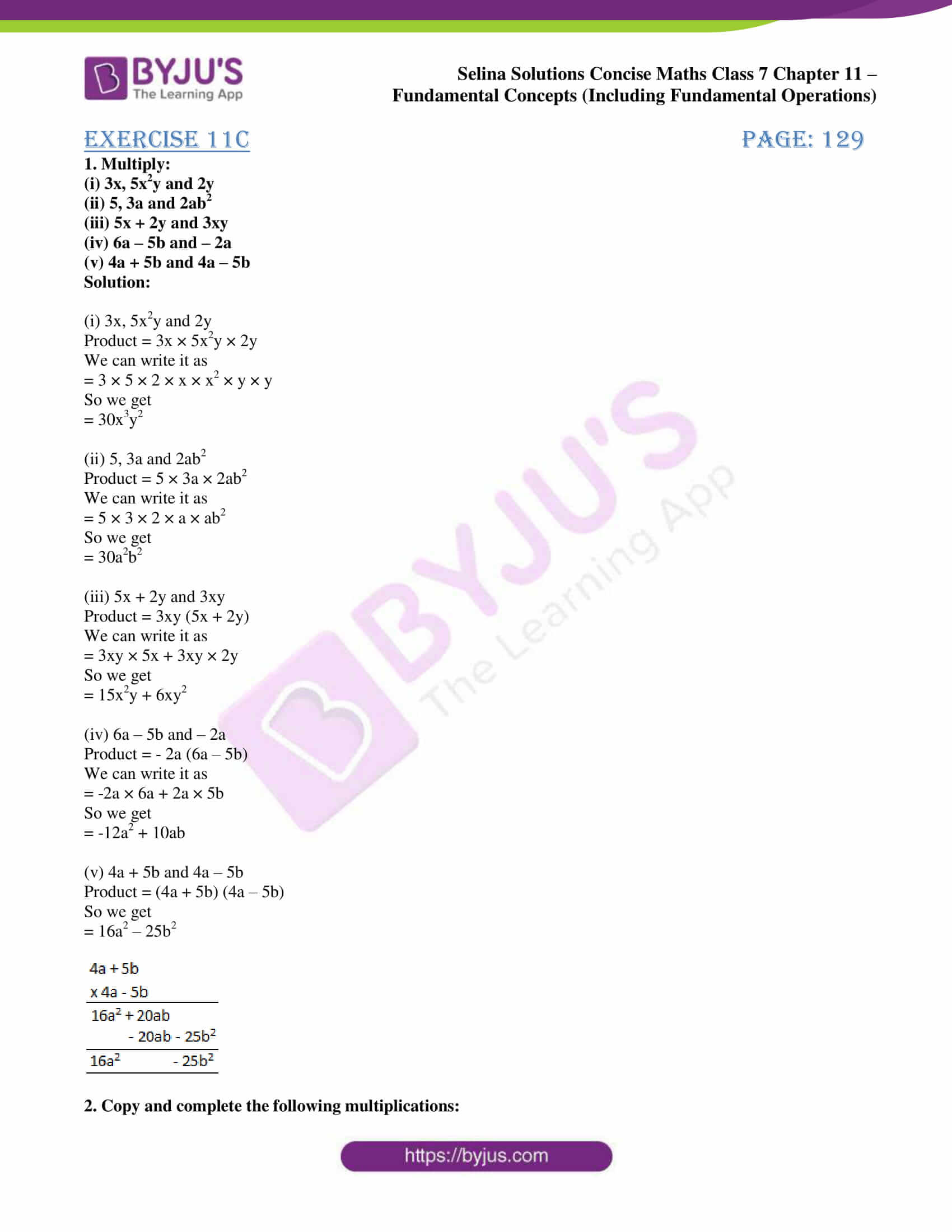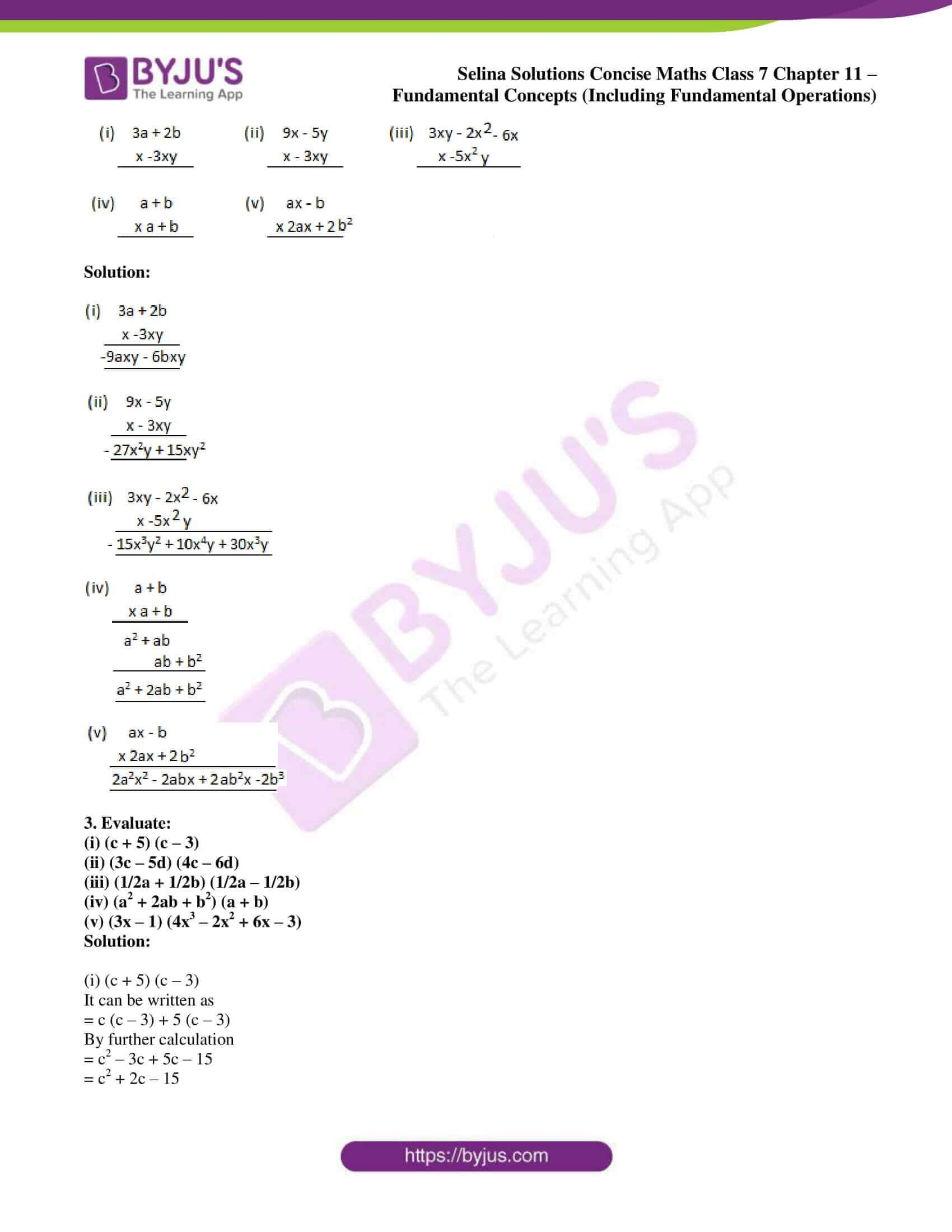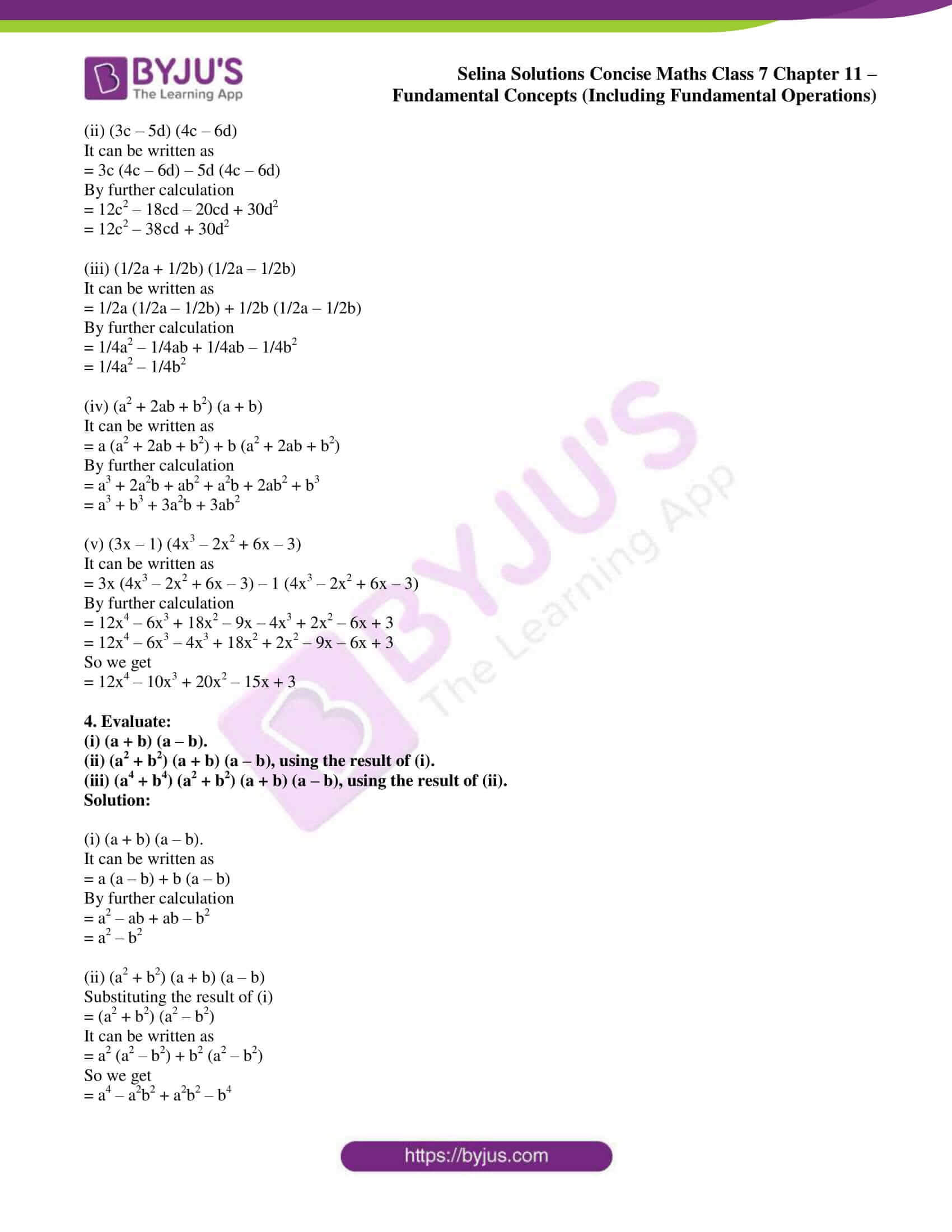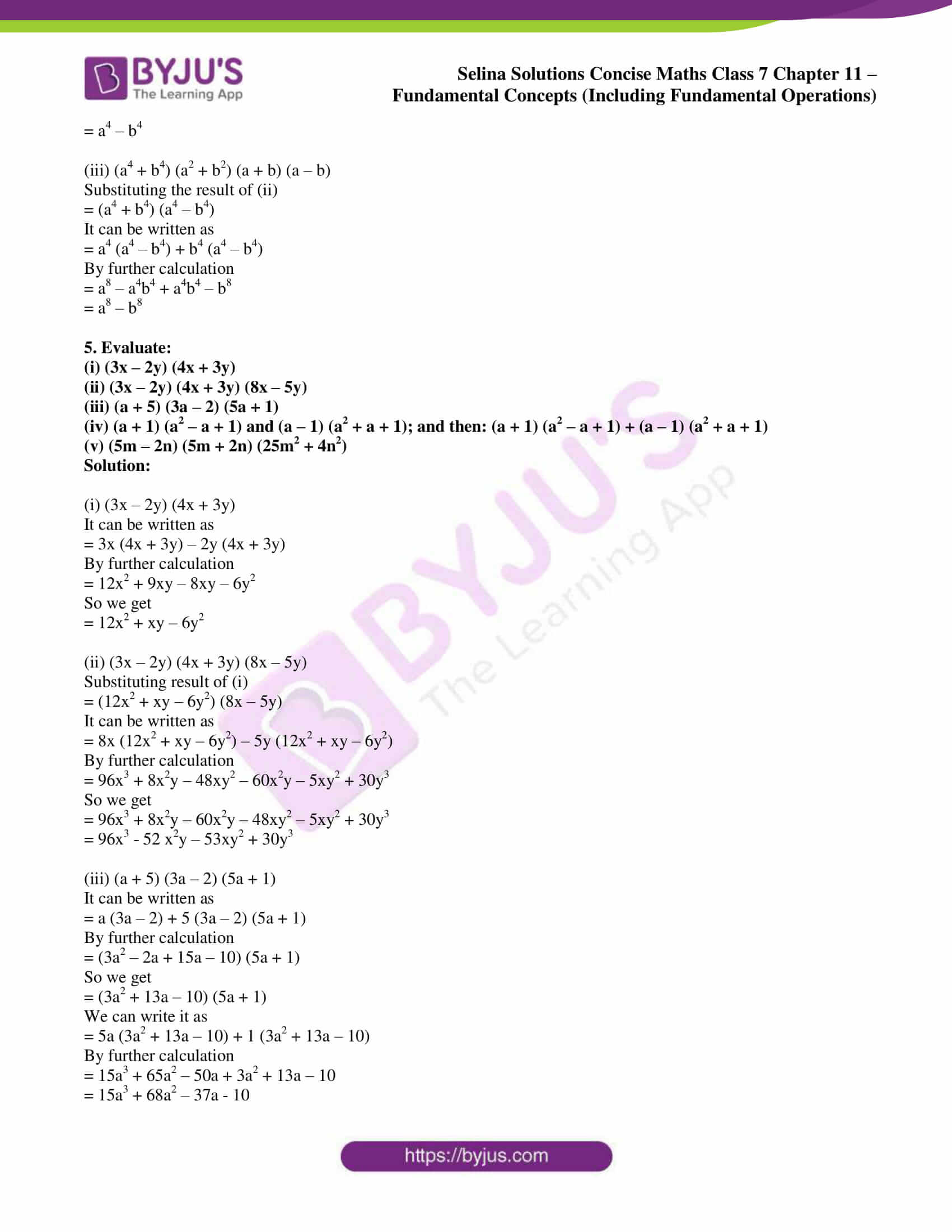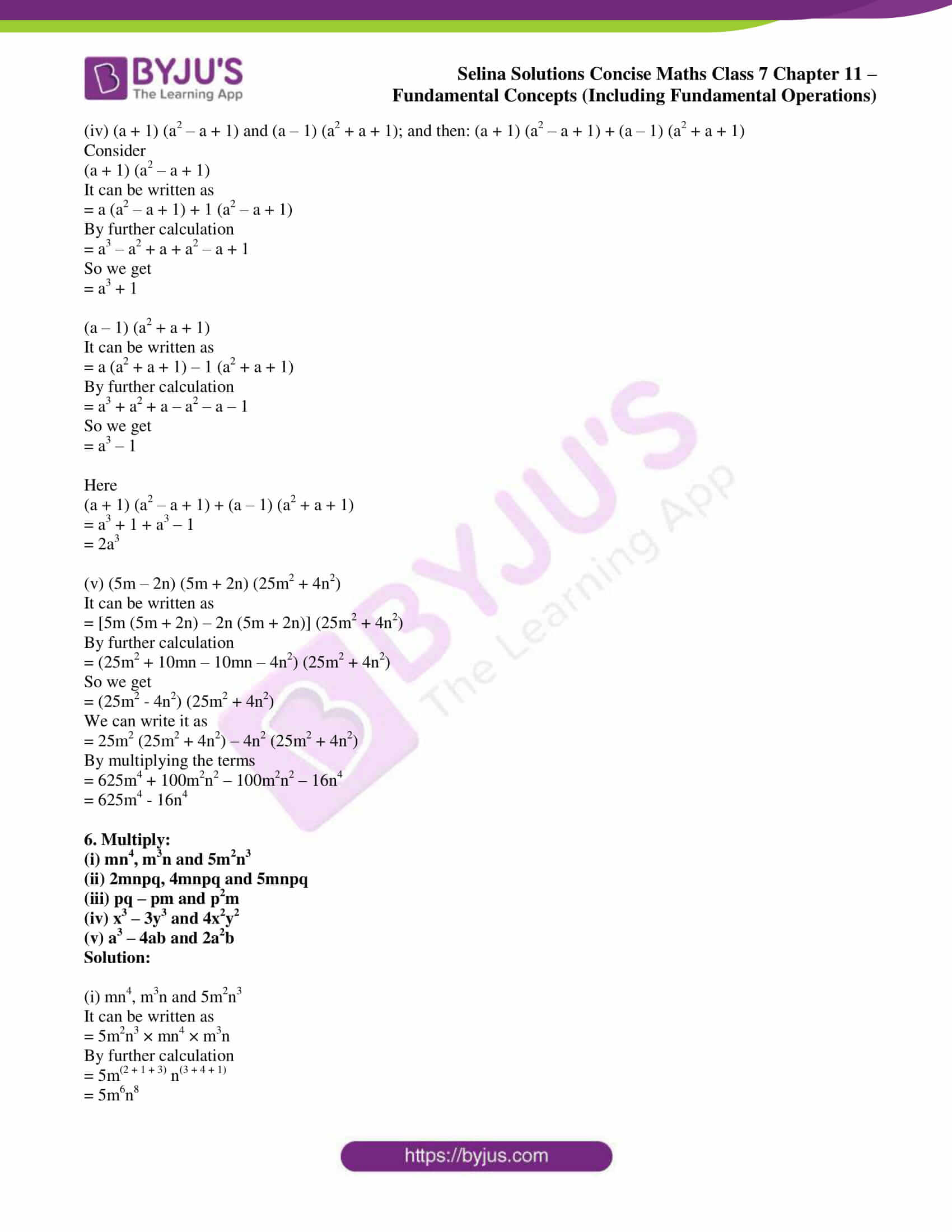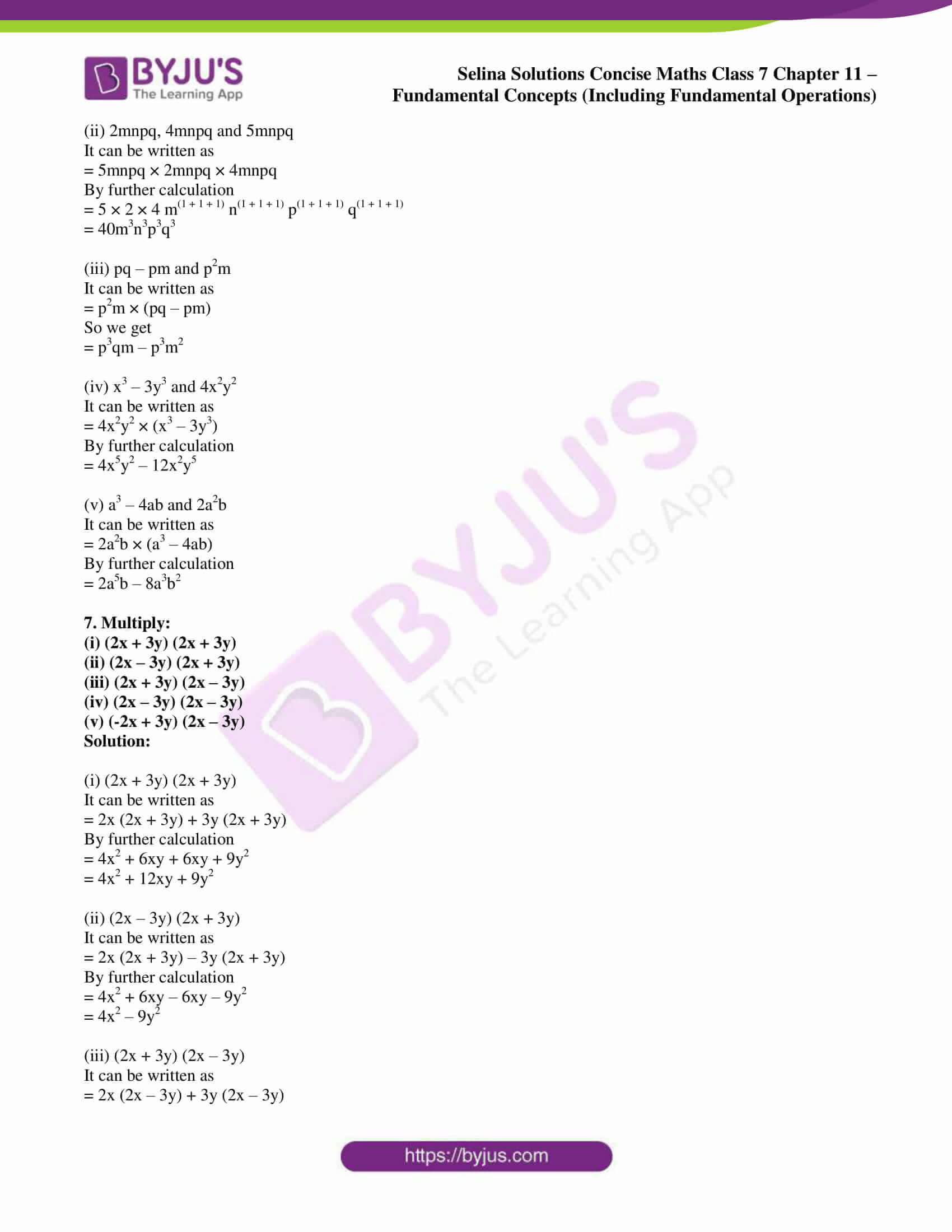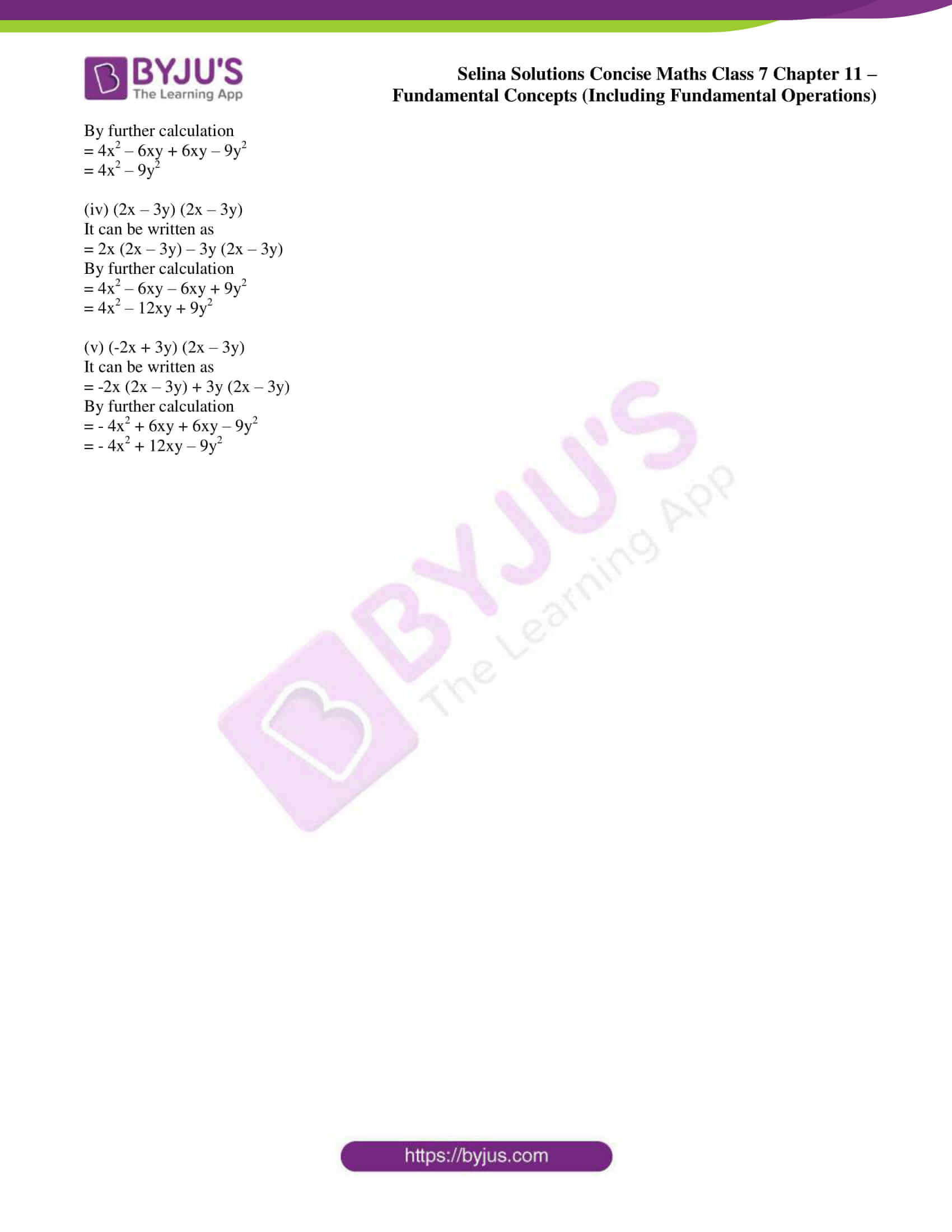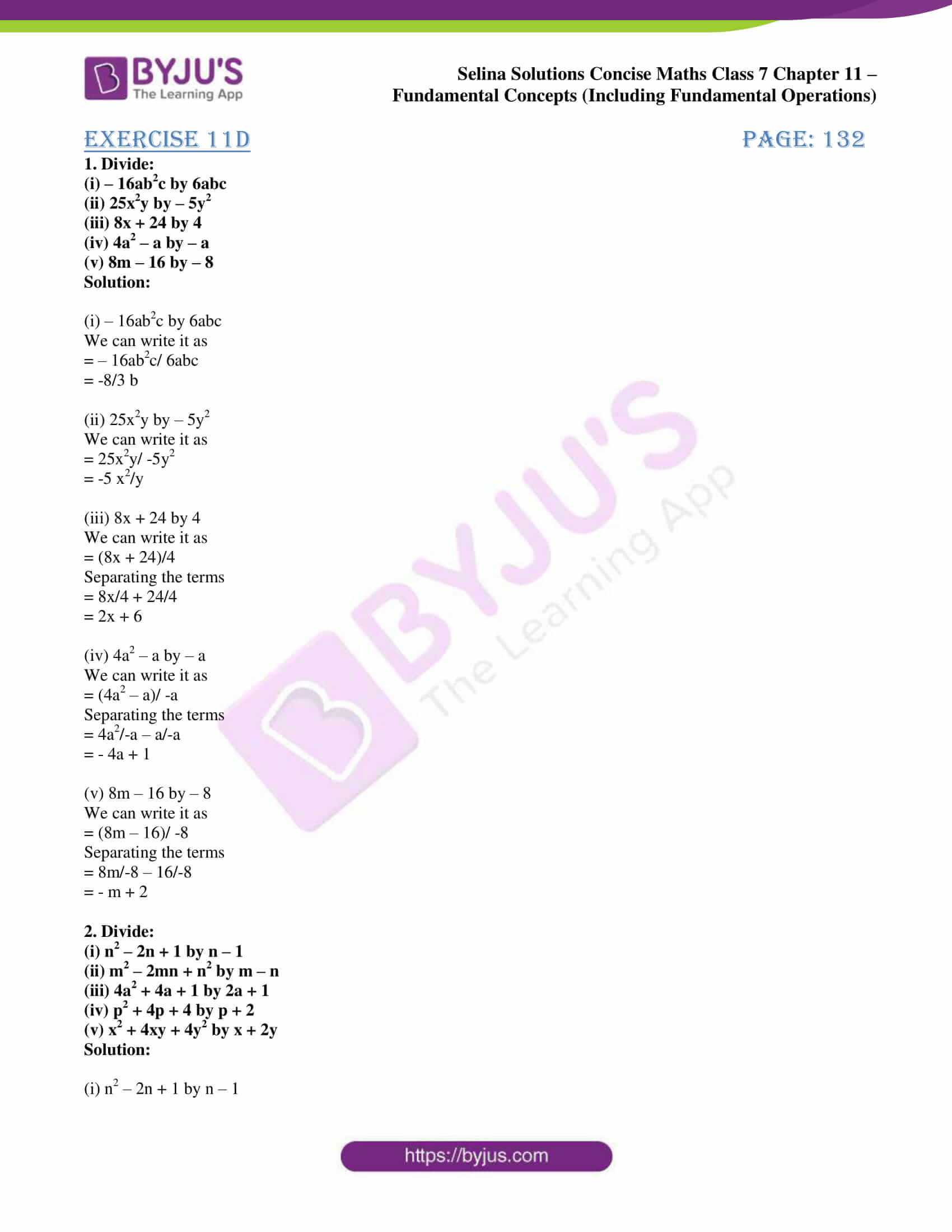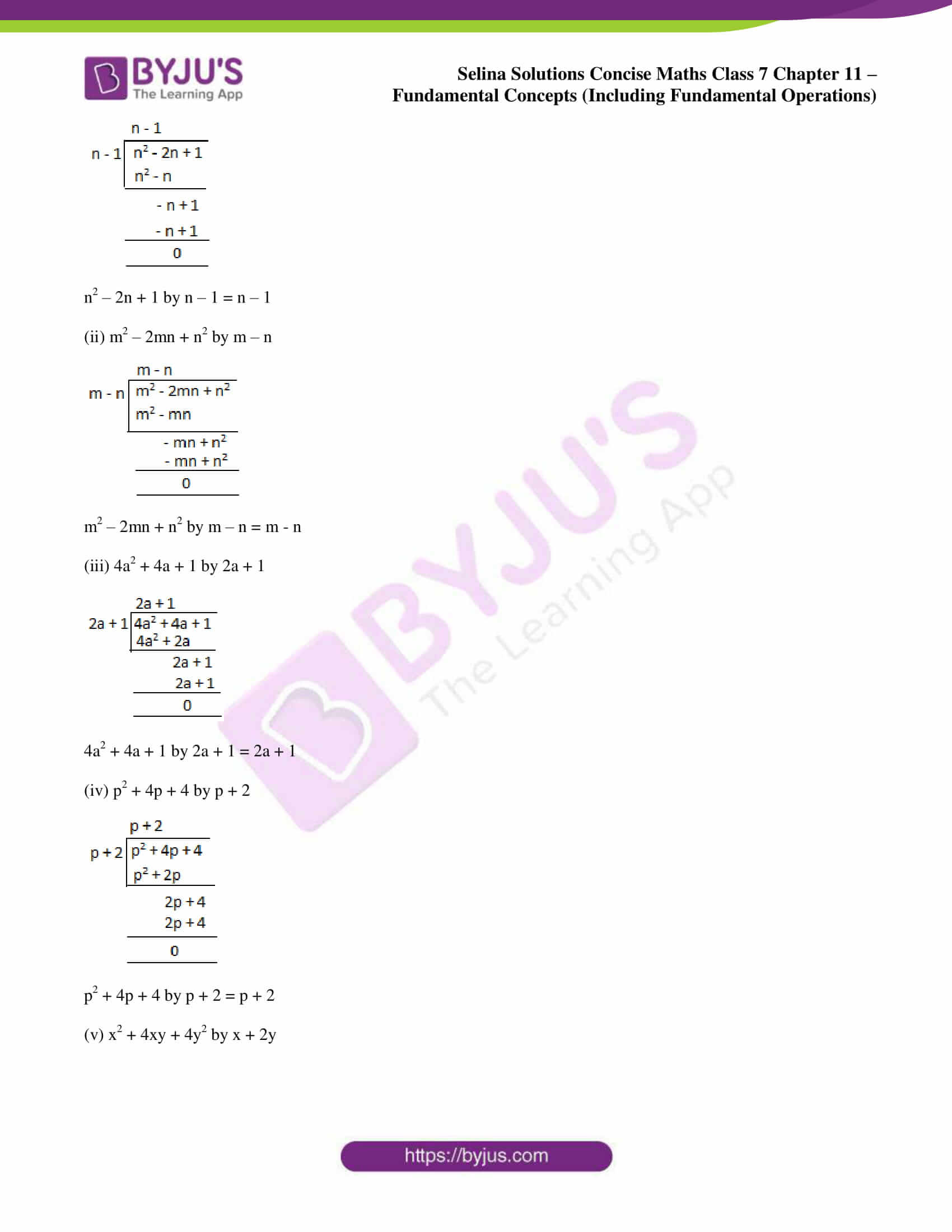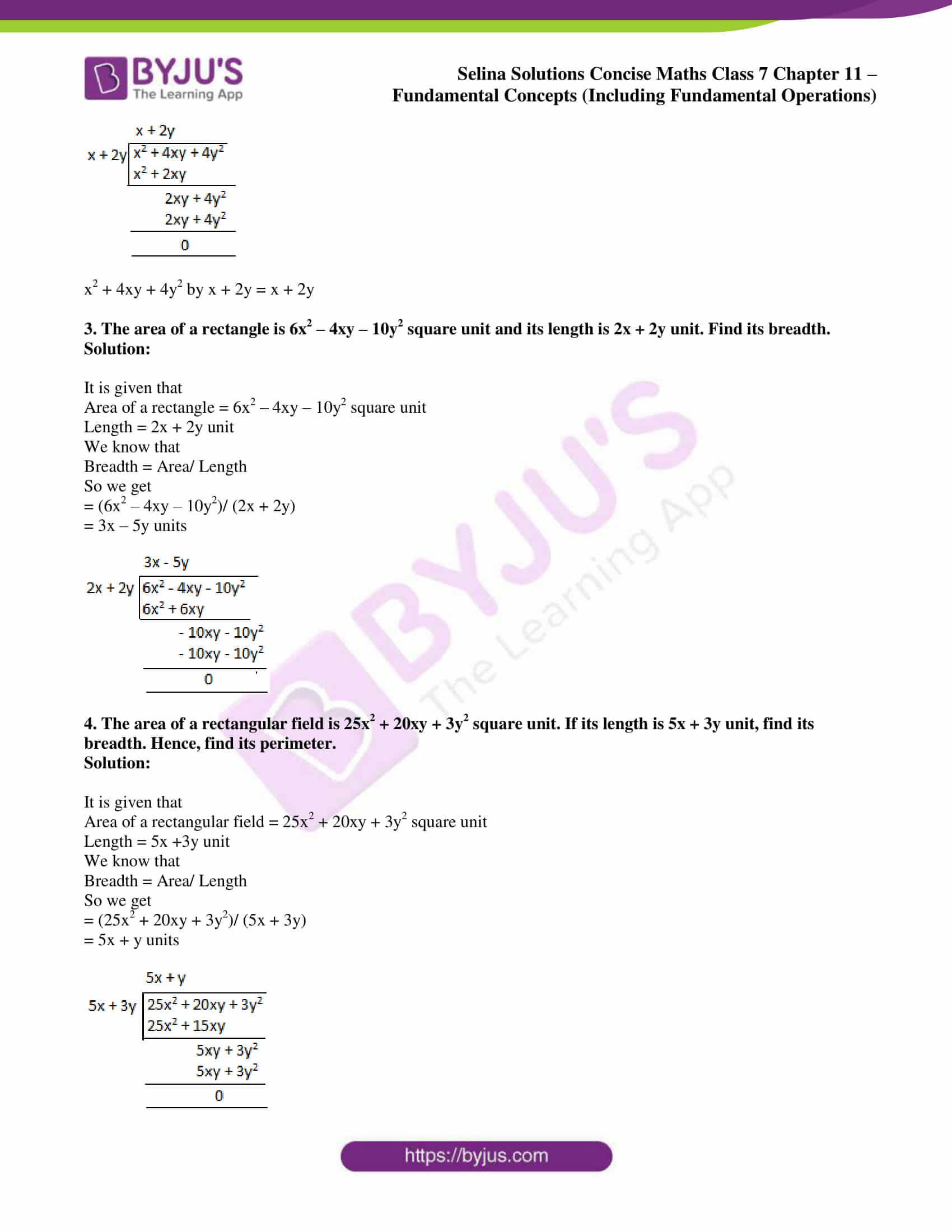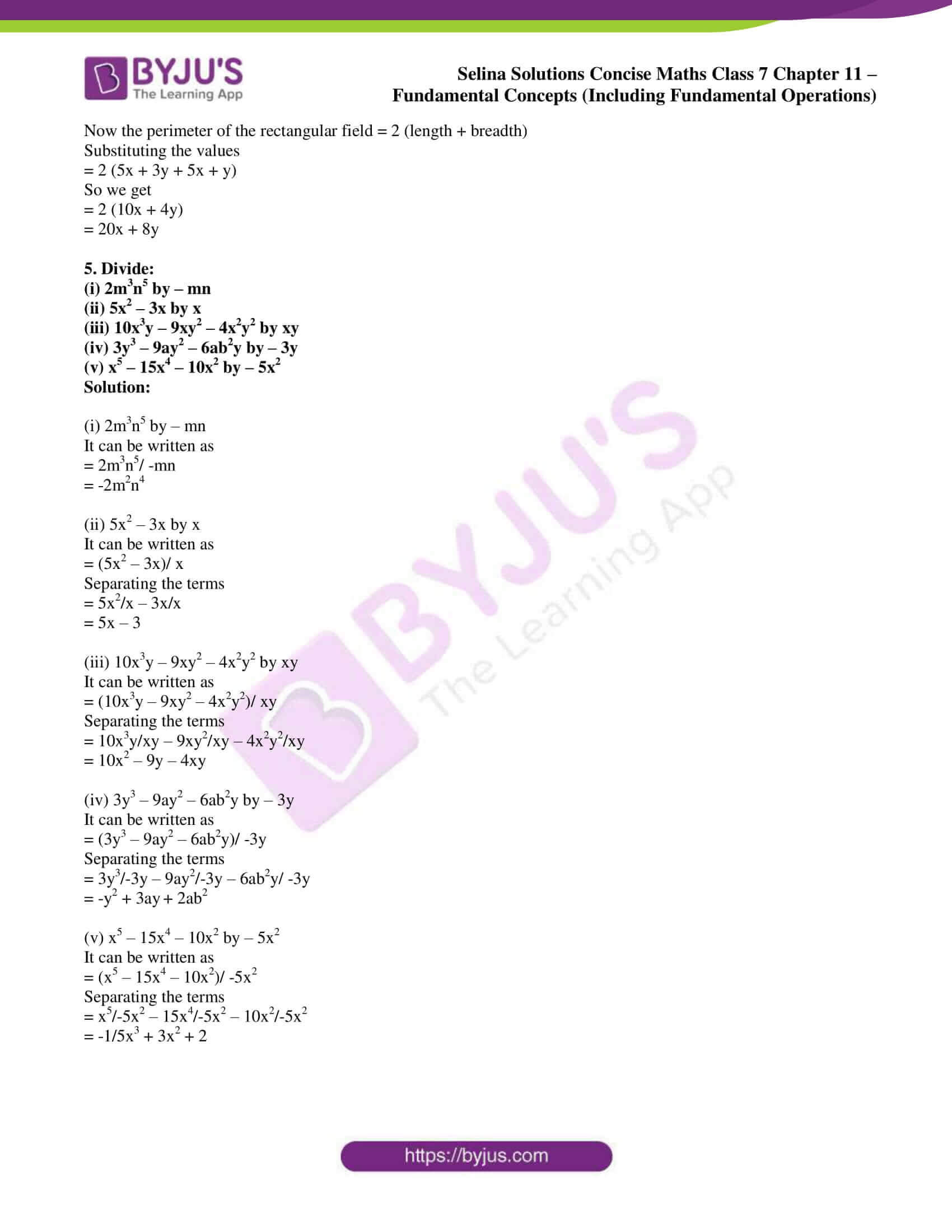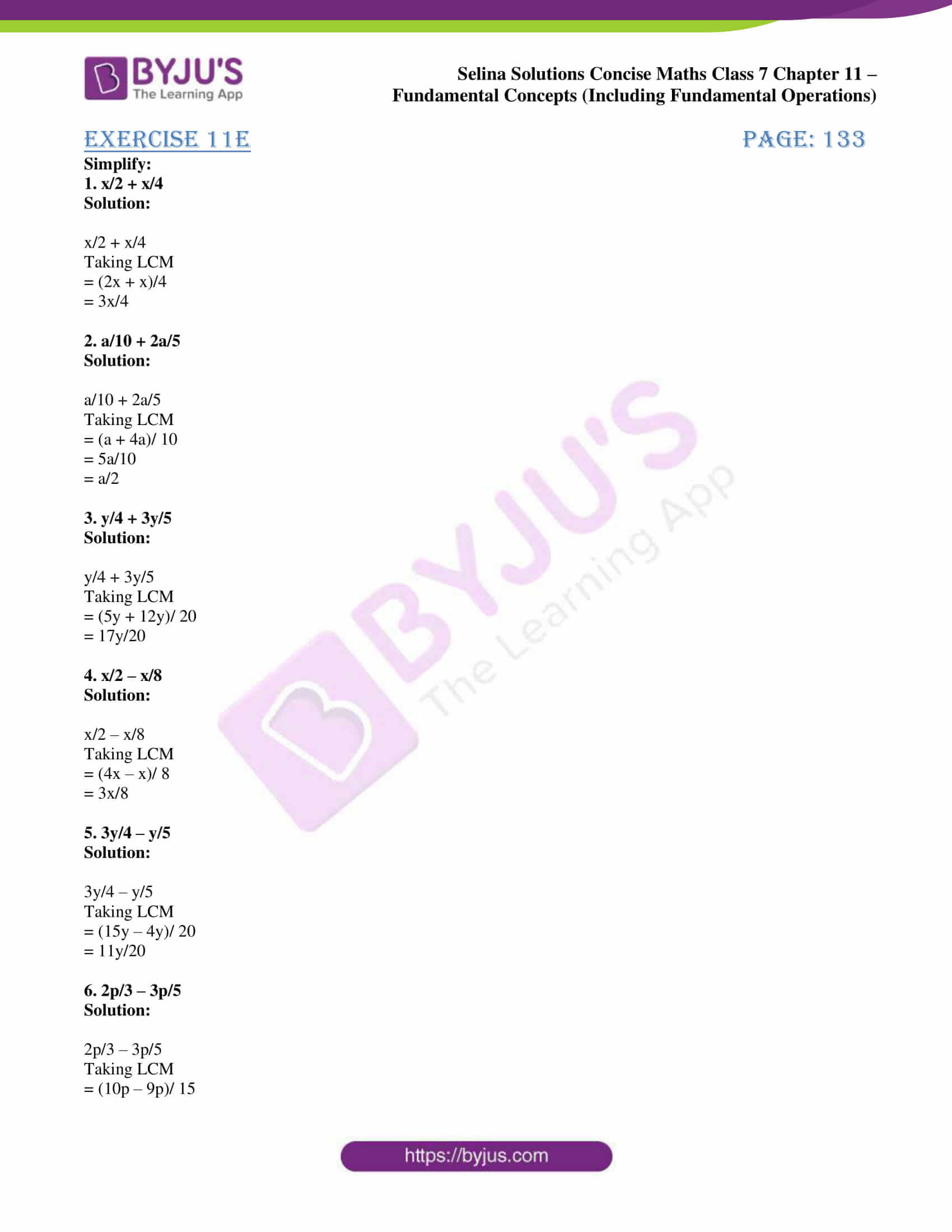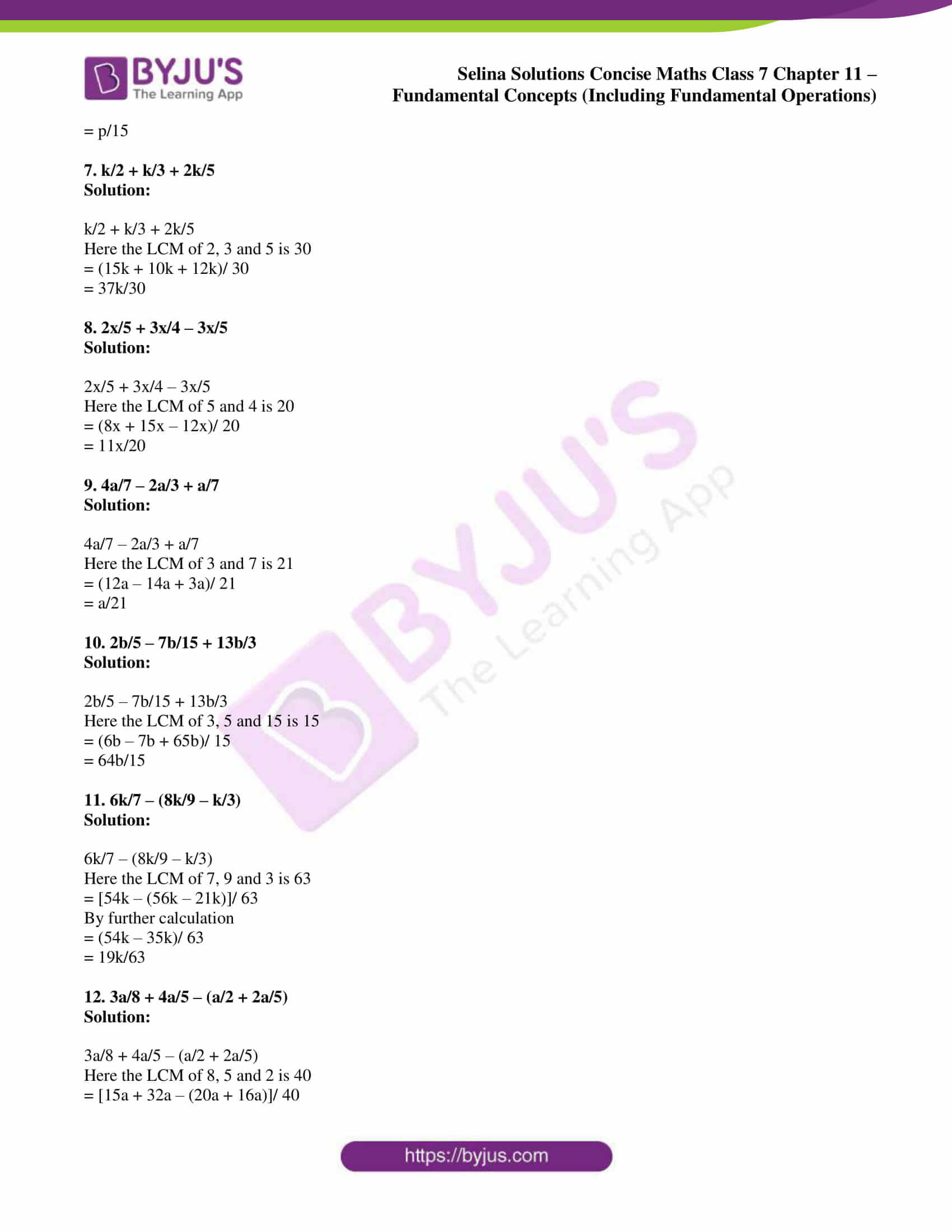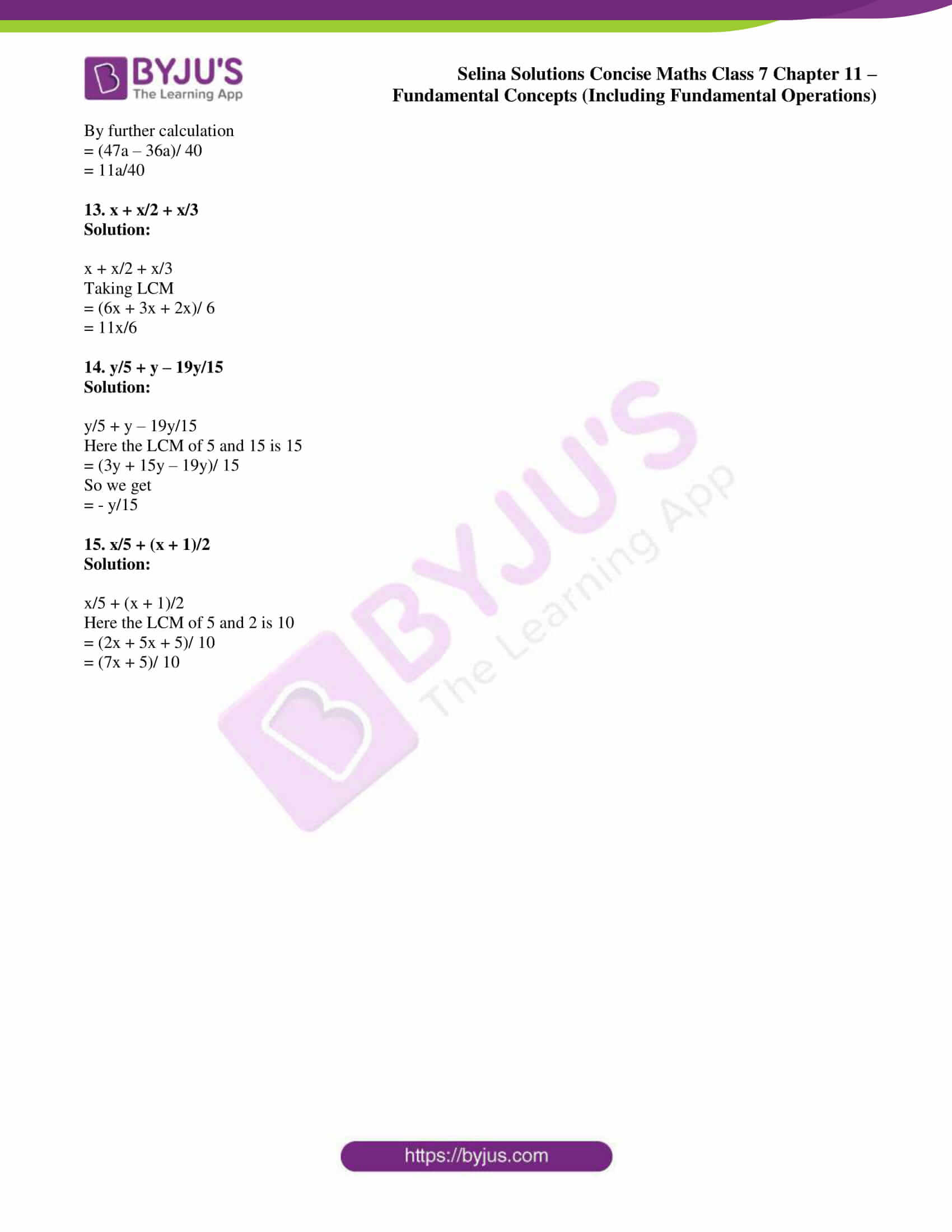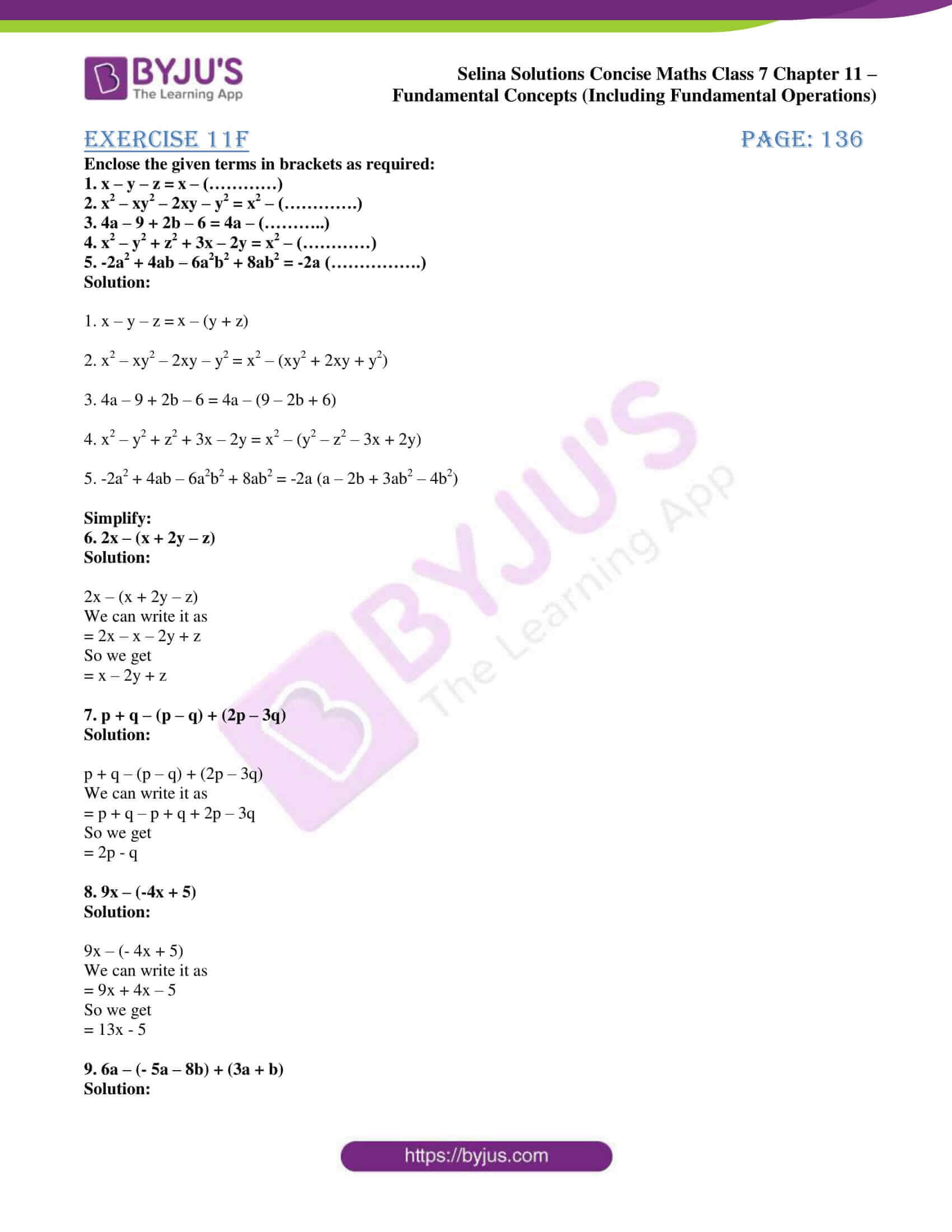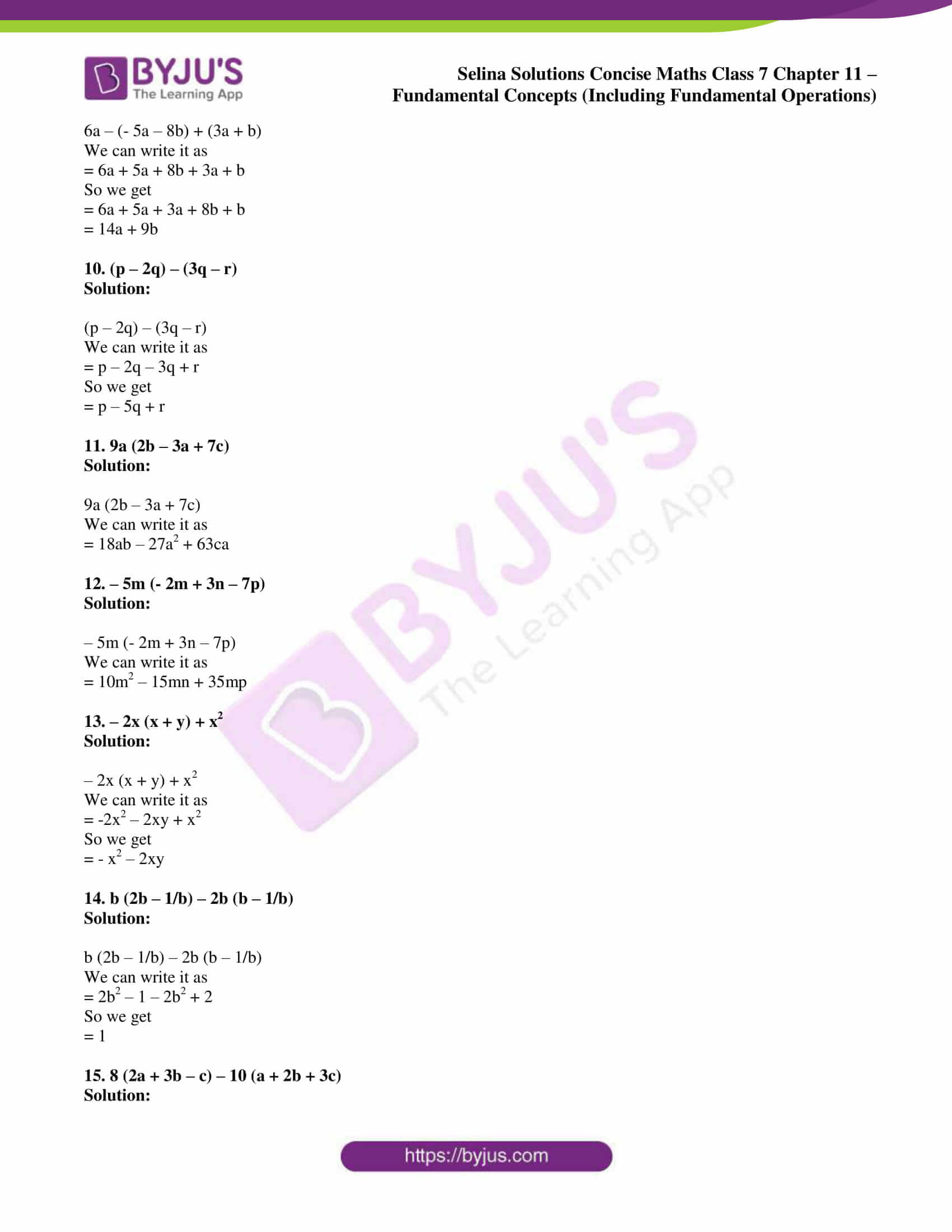### Exercises of Selina Solutions Concise Maths Class 7 Chapter 11 – Fundamental Concepts (Including Fundamental Operations)

Exercise 11A Solutions

Exercise 11B Solutions

Exercise 11C Solutions

Exercise 11D Solutions

Exercise 11E Solutions

Exercise 11F Solutions

## Access Selina Solutions Concise Maths Class 7 Chapter 11: Fundamental Concepts (Including Fundamental Operations)

#### Exercise 11A page: 121

1. Separate constant terms and variable terms from the following:

8, x, 6xy, 6 + x, – 5xy2, 15az2, 32z/ xy, y2/ 3x

Solution:

The constant term is 8.

The variable terms are x, 6xy, 6 + x, – 5xy2, 15az2, 32z/ xy, y2/ 3x.

2. For each expression, given below, state whether it is a monomial, binomial or trinomial:

(i) 2x ÷ 15

(ii) ax + 9

(iii) 3x2 × 5x

(iv) 5 + 2x – 3b

(v) 2y – 7z/3 ÷ x

(vi) 3p × q ÷ z

(vii) 12z ÷ 5x + 4

(viii) 12 – 5z – 4

(ix) a3 -3ab2 × c

Solution:

(i) 2x ÷ 15 = 2x/15

It has one term and hence it is a monomial.

(ii) ax + 9

It has two terms and hence it is a binomial.

(iii) 3x2 × 5x = 15x3

It has one term and hence it is a monomial.

(iv) 5 + 2x – 3b

It has three terms and hence it is a trinomial.

(v) 2y – 7z/3 ÷ x = 2y – 7z/3x

It has two terms and hence it is a binomial.

(vi) 3p × q ÷ z = 3pq/z

It has one term and hence it is a monomial.

(vii) 12z ÷ 5x + 4 = 12z/5x + 4

It has two terms and hence it is a binomial.

(viii) 12 – 5z – 4 = 8 – 5z

It has two terms and hence it is a binomial.

(ix) a3 -3ab2 × c = a3 – 3ab2c

It has two terms and hence it is a binomial.

3. Write the coefficient of:

(i) xy in -3axy

(ii) z2 in p2yz2

(iii) mn in – mn

(iv) 15 in -15p2

Solution:

(i) xy in -3axy

The coefficient of xy in -3axy = -3a

(ii) z2 in p2yz2

The coefficient of z2 in p2yz2 = p2y

(iii) mn in – mn

The coefficient of mn in – mn = -1

(iv) 15 in -15p2

The coefficient of 15 in -15p2 = -p2

4. For each of the following monomials, write its degree:

(i) 7y

(ii) –x2y

(iii) xy2z

(iv) -9y2z3

(v) 3m3n4

(vi) -2p2q3r4

Solution:

(i) The degree of 7y is 1.

(ii) The degree of –x2y = 2 + 1 = 3

(iii) The degree of xy2z = 1 + 2 + 1 = 4

(iv) The degree of -9y2z3 = 2 + 3 = 5

(v) The degree of 3m3n4 = 3 + 4 = 7

(vi) The degree of -2p2q3r4 = 2 + 3 + 4 = 9

5. Write the degree of each of the following polynomials:

(i) 3y3 – x2y2 + 4x

(ii) p3q2 – 6p2q5 + p4q4

(iii) -8mn6 + 5m3n

(iv) 7 – 3x2y + y2

(v) 3x – 15

(vi) 2y2z + 9yz3

Solution:

(i) The degree of 3y3 – x2y2 + 4x is 4

x2y2 is the term which has the highest degree.

(ii) The degree of p3q2 – 6p2q5 + p4q4 is 8

p4q4 is the term which has the highest degree.

(iii) The degree of -8mn6 + 5m3n is 7

-8mn6 is the term which has the highest degree.

(iv) The degree of 7 – 3x2y + y2 is 3

– 3x2y is the term which has the highest degree.

(v) The degree of 3x – 15 is 1

3x is the term which has the highest degree.

(vi) The degree of 2y2z + 9yz3 is 4

9yz3 is the term which has the highest degree.

6. Group the like terms together:

(i) 9x2, xy, -3x2, x2 and -2xy

(ii) ab, -a2b, -3ab, 5a2b and -8a2b.

(iii) 7p, 8pq, -5pq, -2p and 3p

Solution:

(i) 9x2, xy, -3x2, x2 and -2xy

9x2, -3x2 and x2 are like terms

xy and -2xy are like terms.

(ii) ab, -a2b, -3ab, 5a2b and -8a2b

-a2b, 5a2b and -8a2b are like terms

ab and – 3ab are like terms.

(iii) 7p, 8pq, -5pq, -2p and 3p

7p, -2p and 3p are like terms

8pq and -5pq are like terms.

7. Write the numerical coefficient of each of the following:

(i) y

(ii) – y

(iii) 2x2y

(iv) -8xy3

(v) 3py2

(vi) -9a2b3

Solution:

(i) The numerical coefficient of y is 1.

(ii) The numerical coefficient of – y is – 1.

(iii) The numerical coefficient of 2x2y is 2.

(iv) The numerical coefficient of -8xy3 is -8.

(v) The numerical coefficient of 3py2 is 3.

(vi) The numerical coefficient of -9a2b3 is -9.

8. In -5x3y2z4; write the coefficient of:

(i) z2

(ii) y2

(iii) yz2

(iv) x3y

(v) –xy2

(vi) -5xy2z

Also, write the degree of the given algebraic expression.

Solution:

(i) The coefficient of z2 is -5x3y2z2.

(ii) The coefficient of y2 is -5x3z4.

(iii) The coefficient of yz2 is -5x3yz2.

(iv) The coefficient of x3y is -5yz4.

(v) The coefficient of –xy2 is 5x2z4.

(vi) The coefficient of -5xy2z is x2z3.

So the degree of the given algebraic expression = 3 + 2 + 4 = 9.

#### Exercise 11B page: 125

1. Fill in the blanks:

(i) 8x + 5x = …….

(ii) 8x – 5x = ……

(iii) 6xy2 + 9xy2 = …….

(iv) 6xy2 – 9xy2 = …….

(v) The sum of 8a, 6a and 5b = …….

(vi) The addition of 5, 7xy, 6 and 3xy = ……..

(vii) 4a + 3b – 7a + 4b = ……….

(viii) – 15x + 13x + 8 = ……..

(ix) 6x2y + 13xy2 – 4x2y + 2xy2 = ………

(x) 16x2 – 9x2 = ……. and 25xy2 – 17xy2 = ………

Solution:

(i) 8x + 5x = 13x

(ii) 8x – 5x = 3x

(iii) 6xy2 + 9xy2 = 15xy2

(iv) 6xy2 – 9xy2 = -3xy2

(v) The sum of 8a, 6a and 5b = 14a + 5b

It can be written as

8a + 6a + 5b = 14a + 5b

(vi) The addition of 5, 7xy, 6 and 3xy = 11 + 10xy

It can be written as

5 + 7xy + 6 + 3xy = 11 + 10xy

(vii) 4a + 3b – 7a + 4b = 7b – 3a

It can be written as

4a + 3b – 7a + 4b = (4 – 7)a + (3 + 4)b

= -3a + 7b

(viii) – 15x + 13x + 8 = 8 – 2x

It can be written as

-15x + 13x + 8 = (-15 + 13) x + 8 = -2x + 8

(ix) 6x2y + 13xy2 – 4x2y + 2xy2 = 2x2y + 15xy2

It can be written as

6x2y + 13xy2 – 4x2y + 2xy2 = (6 – 4) x2y + (13 + 2) xy2

= 2x2y + 15xy2

(x) 16x2 – 9x2 = 7x2 and 25xy2 – 17xy2 = 8xy2

(i) -9x, 3x and 4x

(ii) 23y2, 8y2 and – 12y2

(iii) 18pq, -15pq and 3pq

Solution:

(i) -9x, 3x and 4x

It can be written as

= -9x + 3x + 4x

So we get

= -9x + 7x

= -2x

(ii) 23y2, 8y2 and – 12y2

It can be written as

= 23y2 + 8y2 – 12y2

So we get

= 31y2 – 12y2

= 19y2

(iii) 18pq, -15pq and 3pq

It can be written as

= 18pq – 15pq + 3pq

So we get

= 3pq + 3pq

= 6pq

3. Simplify:

(i) 3m + 12m – 5m

(ii) 7n2 – 9n2 + 3n2

(iii) 25zy – 8zy – 6zy

(iv) -5ax2 + 7ax2 – 12ax2

(v) – 16am + 4mx + 4am – 15mx + 5am

Solution:

(i) 3m + 12m – 5m

It can be written as

= 15m – 5m

So we get

= 10m

(ii) 7n2 – 9n2 + 3n2

It can be written as

= (7 + 3) n2 – 9n2

So we get

= 10n2 – 9n2

= n2

(iii) 25zy – 8zy – 6zy

It can be written as

= 25zy – 14zy

So we get

= 11zy

(iv) -5ax2 + 7ax2 – 12ax2

It can be written as

= (-5 – 12) ax2 + 7ax2

So we get

= -17ax2 + 7ax2

= -10ax2

(v) – 16am + 4mx + 4am – 15mx + 5am

It can be written as

= (-16 + 4 + 5) am + (4 – 15) mx

So we get

= – 7am – 11mx

(i) a + b and 2a + 3b

(ii) 2x + y and 3x – 4y

(iii) -3a + 2b and 3a + b

(iv) 4 + x, 5 – 2x and 6x

Solution:

(i) a + b and 2a + 3b

It can be written as

= a + b + 2a + 3b

So we get

= a + 2a + b + 3b

= 3a + 4b

(ii) 2x + y and 3x – 4y

It can be written as

= 2x + y + 3x – 4y

So we get

= 2x + 3x + y – 4y

= 5x – 3y

(iii) -3a + 2b and 3a + b

It can be written as

= -3a + 2b + 3a + b

So we get

= -3a + 3a + 2b + b

= 3b

(iv) 4 + x, 5 – 2x and 6x

It can be written as

= 4 + x + 5 – 2x + 6x

So we get

= x – 2x + 6x + 4 + 5

= 5x + 9

5. Find the sum of:

(i) 3x + 8y + 7z, 6y + 4z – 2x and 3y – 4x + 6z

(ii) 3a + 5b + 2c, 2a + 3b – c and a + b + c

(iii) 4x2 + 8xy – 2y2 and 8xy – 5y2 + x2

(iv) 9x2 – 6x + 7, 5 – 4x and 6 – 3x2

(v) 5x2 – 2xy + 3y2, -2x2 + 5xy + 9y2 and 3x2 – xy – 4y2

Solution:

(i) 3x + 8y + 7z, 6y + 4z – 2x and 3y – 4x + 6z

It can be written as

= 3x + 8y + 7z + 6y + 4z – 2x + 3y – 4x + 6z

By further calculation

= 3x – 2x – 4x + 8y + 6y + 3y + 7z + 4z + 6z

So we get

= 3x – 6x + 17y + 17z

= -3x + 17y + 17z

(ii) 3a + 5b + 2c, 2a + 3b – c and a + b + c

It can be written as

= 3a + 5b + 2c + 2a + 3b – c + a + b + c

By further calculation

= 3a + 2a + a + 5b + 3b + b + 2c – c + c

So we get

= 6a + 9b + 3c – c

= 6a + 9b + 2c

(iii) 4x2 + 8xy – 2y2 and 8xy – 5y2 + x2

It can be written as

= 4x2 + 8xy – 2y2 + 8xy – 5y2 + x2

By further calculation

= 4x2 + x2 + 8xy + 8xy – 2y2 – 5y2

So we get

= 5x2 + 16xy – 7y2

(iv) 9x2 – 6x + 7, 5 – 4x and 6 – 3x2

It can be written as

= 9x2 – 6x + 7 + 5 – 4x + 6 – 3x2

By further calculation

= 9x2 – 3x2 – 6x – 4x + 7 + 5 + 6

So we get

= 6x2 – 10x + 18

(v) 5x2 – 2xy + 3y2, -2x2 + 5xy + 9y2 and 3x2 – xy – 4y2

It can be written as

= 5x2 – 2xy + 3y2 – 2x2 + 5xy + 9y2 + 3x2 – xy – 4y2

By further calculation

= 5x2 – 2x2 + 3x2 – 2xy + 5xy – xy + 3y2 + 9y2 – 4y2

So we get

= 6x2 + 2xy + 8y2

6. Find the sum of:

(i) x and 3y

(ii) -2a and +5

(iii) -4x2 and + 7x

(iv) +4a and -7b

(v) x3, 3x2y and 2y2

(vi) 11 and –by

Solution:

(i) x and 3y

The sum of x and 3y is x + 3y.

(ii) -2a and +5

The sum of -2a and + 5 is -2a + 5.

(iii) -4x2 and + 7x

The sum of -4x2 and + 7x is -4x2 + 7x.

(iv) +4a and -7b

The sum of +4a and -7b is + 4a – 7b.

(v) x3, 3x2y and 2y2

The sum of x3, 3x2y and 2y2 is x3 + 3x2y + 2y2.

(vi) 11 and –by

The sum of 11 and -by is 11 – by.

7. The sides of a triangle are 2x + 3y, x + 5y and 7x -2y. Find its perimeter.

Solution:

It is given that

Sides of a triangle are 2x + 3y, x + 5y and 7x -2y

We know that

Perimeter = Sum of all three sides of a triangle

Substituting the values

= 2x + 3y + x + 5y + 7x – 2y

By further calculation

= 2x + x + 7x + 3y + 5y – 2y

So we get

= 10x + 8y – 2y

= 10x + 6y

8. The two adjacent sides of a rectangle are 6a + 9b and 8a – 4b. Find its perimeter.

Solution:

It is given that

Sides of a rectangle are 6a + 9b and 8a – 4b

So length = 6a + 9b and breadth = 8a – 4b

We know that

Perimeter = 2 (length + breadth)

Substituting the values

= 2 (6a + 9b + 8a – 4b)

By further calculation

= 2 (14a + 5b)

So we get

= 28a + 10b

9. Subtract the second expression from the first:

(i) 2a + b, a + b

(ii) -2b + 2c, b + 3c

(iii) 5a + b, -6b + 2a

(iv) a3 – 1 + a, 3a – 2a2

(v) p + 2, 1

Solution:

(i) 2a + b, a + b

It can be written as

= (2a + b) – (a + b)

So we get

= 2a + b – a – b

= 2a – a + b – b

= a

(ii) -2b + 2c, b + 3c

It can be written as

= (-2b + 2c) – (b + 3c)

So we get

= -2b + 2c – b – 3c

= -2b – b + 2c – 3c

= -3b – c

(iii) 5a + b, -6b + 2a

It can be written as

= (5a + b) – (-6b + 2a)

So we get

= 5a + b + 6b – 2a

= 5a – 2a + b + 6b

= 3a + 7b

(iv) a3 – 1 + a, 3a – 2a2

It can be written as

= (a3 – 1 + a) – (3a – 2a2)

So we get

= a3 – 1 + a – 3a + 2a2

= a3 + 2a2 + a – 3a – 1

= a3 + 2a2 – 2a – 1

(v) p + 2, 1

It can be written as

= p + 2 – 1

So we get

= p + 1

10. Subtract:

(i) 4x from 8 – x

(ii) -8c from c + 3d

(iii) – 5a – 2b from b + 6c

(iv) 4p + p2 from 3p2 – 8p

(v) 5a – 3b + 2c from 4a – b – 2c

Solution:

(i) 4x from 8 – x

It can be written as

= (8 – x) – 4x

By further calculation

= 8 – x – 4x

= 8 – 5x

(ii) -8c from c + 3d

It can be written as

= (c + 3d) – (-8c)

By further calculation

= c + 3d + 8c

= 9c + 3d

(iii) – 5a – 2b from b + 6c

It can be written as

= (b + 6c) – (-5a – 2b)

By further calculation

= b + 6c + 5a + 2b

= 5a + 3b + 6c

(iv) 4p + p2 from 3p2 – 8p

It can be written as

= (3p2 – 8p) – (4p + p2)

By further calculation

= 3p2 – 8p – 4p – p2

= 2p2 – 12p

(v) 5a – 3b + 2c from 4a – b – 2c

It can be written as

= (4a – b – 2c) – (5a – 3b + 2c)

By further calculation

= 4a – b – 2c – 5a + 3b – 2c

= -a + 2b – 4c

11. Subtract -5a2 – 3a + 1 from the sum of 4a2 + 3 – 8a and 9a – 7.

Solution:

We know that

Sum of 4a2 + 3 – 8a and 9a – 7 can be written as

= 4a2 + 3 – 8a + 9a – 7

By further calculation

= 4a2 + a – 4

Here

(4a2 + a – 4) – (-5a2 – 3a + 1) = 4a2 + a – 4 + 5a2 + 3a – 1

By further calculation

= 4a2 + 5a2 + a + 3a – 4 – 1

So we get

= 9a2 + 4a – 5

12. By how much does 8x3 – 6x2 + 9x – 10 exceed 4x3 + 2x2 + 7x – 3?

Solution:

We know that

8x3 – 6x2 + 9x – 10 exceed 4x3 + 2x2 + 7x – 3

It can be written as

= (8x3 – 6x2 + 9x – 10) – (4x3 + 2x2 + 7x – 3)

By further calculation

= 8x3 – 6x2 + 9x – 10 – 4x3 – 2x2 -7x + 3

So we get

= 8x3 – 4x3 – 6x2 – 2x2 + 9x – 7x – 10 + 3

= 4x3 – 8x2 + 2x – 7

13. What must be added to 2a3 + 5a – a2 – 6 to get a2 – a – a3 + 1?

Solution:

The answer can be obtained by subtracting 2a3 + 5a – a2 – 6 from a2 – a – a3 + 1

= (-a3 + a2 – a + 1) – (2a3 + 5a – a2 – 6)

It can be written as

= -a3 + a2 – a + 1 – 2a3 – 5a + a2 + 6

By further calculation

= – a3 – 2a3 + a2 + a2 – a – 5a + 1 + 6

= -3a3 + 2a2 – 6a + 7

14. What must be subtracted from a2 + b2 + 2ab to get – 4ab + 2b2?

Solution:

The answer can be obtained by subtracting – 4ab + 2b2 from a2 + b2 + 2ab

= a2 + b2 + 2ab – (– 4ab + 2b2)

It can be written as

= a2 + b2 + 2ab + 4ab – 2b2

By further calculation

= a2 + b2 – 2b2 + 2ab + 4ab

= a2 – b2 + 6ab

15. Find the excess of 4m2 + 4n2 + 4p2 over m2 + 3n2 – 5p2.

Solution:

The answer can be obtained by subtracting m2 + 3n2 – 5p2 from 4m2 + 4n2 + 4p2

= (4m2 + 4n2 + 4p2) – (m2 + 3n2 – 5p2)

It can be written as

= 4m2 + 4n2 + 4p2 – m2 – 3n2 + 5p2

By further calculation

= 4m2 – m2 + 4n2 – 3n2 + 4p2 + 5p2

= 3m2 + n2 + 9p2

#### Exercise 11C page: 129

1. Multiply:

(i) 3x, 5x2y and 2y

(ii) 5, 3a and 2ab2

(iii) 5x + 2y and 3xy

(iv) 6a – 5b and – 2a

(v) 4a + 5b and 4a – 5b

Solution:

(i) 3x, 5x2y and 2y

Product = 3x × 5x2y × 2y

We can write it as

= 3 × 5 × 2 × x × x2 × y × y

So we get

= 30x3y2

(ii) 5, 3a and 2ab2

Product = 5 × 3a × 2ab2

We can write it as

= 5 × 3 × 2 × a × ab2

So we get

= 30a2b2

(iii) 5x + 2y and 3xy

Product = 3xy (5x + 2y)

We can write it as

= 3xy × 5x + 3xy × 2y

So we get

= 15x2y + 6xy2

(iv) 6a – 5b and – 2a

Product = – 2a (6a – 5b)

We can write it as

= -2a × 6a + 2a × 5b

So we get

= -12a2 + 10ab

(v) 4a + 5b and 4a – 5b

Product = (4a + 5b) (4a – 5b)

So we get

= 16a2 – 25b2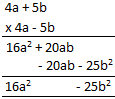2. Copy and complete the following multiplications:Solution: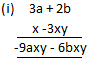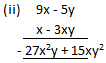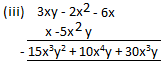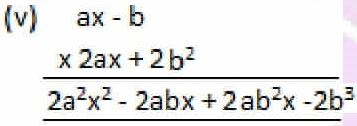3. Evaluate:

(i) (c + 5) (c – 3)

(ii) (3c – 5d) (4c – 6d)

(iii) (1/2a + 1/2b) (1/2a – 1/2b)

(iv) (a2 + 2ab + b2) (a + b)

(v) (3x – 1) (4x3 – 2x2 + 6x – 3)

Solution:

(i) (c + 5) (c – 3)

It can be written as

= c (c – 3) + 5 (c – 3)

By further calculation

= c2 – 3c + 5c – 15

= c2 + 2c – 15

(ii) (3c – 5d) (4c – 6d)

It can be written as

= 3c (4c – 6d) – 5d (4c – 6d)

By further calculation

= 12c2 – 18cd – 20cd + 30d2

= 12c2 – 38cd + 30d2

(iii) (1/2a + 1/2b) (1/2a – 1/2b)

It can be written as

= 1/2a (1/2a – 1/2b) + 1/2b (1/2a – 1/2b)

By further calculation

= 1/4a2 – 1/4ab + 1/4ab – 1/4b2

= 1/4a2 – 1/4b2

(iv) (a2 + 2ab + b2) (a + b)

It can be written as

= a (a2 + 2ab + b2) + b (a2 + 2ab + b2)

By further calculation

= a3 + 2a2b + ab2 + a2b + 2ab2 + b3

= a3 + b3 + 3a2b + 3ab2

(v) (3x – 1) (4x3 – 2x2 + 6x – 3)

It can be written as

= 3x (4x3 – 2x2 + 6x – 3) – 1 (4x3 – 2x2 + 6x – 3)

By further calculation

= 12x4 – 6x3 + 18x2 – 9x – 4x3 + 2x2 – 6x + 3

= 12x4 – 6x3 – 4x3 + 18x2 + 2x2 – 9x – 6x + 3

So we get

= 12x4 – 10x3 + 20x2 – 15x + 3

4. Evaluate:

(i) (a + b) (a – b).

(ii) (a2 + b2) (a + b) (a – b), using the result of (i).

(iii) (a4 + b4) (a2 + b2) (a + b) (a – b), using the result of (ii).

Solution:

(i) (a + b) (a – b).

It can be written as

= a (a – b) + b (a – b)

By further calculation

= a2 – ab + ab – b2

= a2 – b2

(ii) (a2 + b2) (a + b) (a – b)

Substituting the result of (i)

= (a2 + b2) (a2 – b2)

It can be written as

= a2 (a2 – b2) + b2 (a2 – b2)

So we get

= a4 – a2b2 + a2b2 – b4

= a4 – b4

(iii) (a4 + b4) (a2 + b2) (a + b) (a – b)

Substituting the result of (ii)

= (a4 + b4) (a4 – b4)

It can be written as

= a4 (a4 – b4) + b4 (a4 – b4)

By further calculation

= a8 – a4b4 + a4b4 – b8

= a8 – b8

5. Evaluate:

(i) (3x – 2y) (4x + 3y)

(ii) (3x – 2y) (4x + 3y) (8x – 5y)

(iii) (a + 5) (3a – 2) (5a + 1)

(iv) (a + 1) (a2 – a + 1) and (a – 1) (a2 + a + 1); and then: (a + 1) (a2 – a + 1) + (a – 1) (a2 + a + 1)

(v) (5m – 2n) (5m + 2n) (25m2 + 4n2)

Solution:

(i) (3x – 2y) (4x + 3y)

It can be written as

= 3x (4x + 3y) – 2y (4x + 3y)

By further calculation

= 12x2 + 9xy – 8xy – 6y2

So we get

= 12x2 + xy – 6y2

(ii) (3x – 2y) (4x + 3y) (8x – 5y)

Substituting result of (i)

= (12x2 + xy – 6y2) (8x – 5y)

It can be written as

= 8x (12x2 + xy – 6y2) – 5y (12x2 + xy – 6y2)

By further calculation

= 96x3 + 8x2y – 48xy2 – 60x2y – 5xy2 + 30y3

So we get

= 96x3 + 8x2y – 60x2y – 48xy2 – 5xy2 + 30y3

= 96x3 – 52 x2y – 53xy2 + 30y3

(iii) (a + 5) (3a – 2) (5a + 1)

It can be written as

= a (3a – 2) + 5 (3a – 2) (5a + 1)

By further calculation

= (3a2 – 2a + 15a – 10) (5a + 1)

So we get

= (3a2 + 13a – 10) (5a + 1)

We can write it as

= 5a (3a2 + 13a – 10) + 1 (3a2 + 13a – 10)

By further calculation

= 15a3 + 65a2 – 50a + 3a2 + 13a – 10

= 15a3 + 68a2 – 37a – 10

(iv) (a + 1) (a2 – a + 1) and (a – 1) (a2 + a + 1); and then: (a + 1) (a2 – a + 1) + (a – 1) (a2 + a + 1)

Consider

(a + 1) (a2 – a + 1)

It can be written as

= a (a2 – a + 1) + 1 (a2 – a + 1)

By further calculation

= a3 – a2 + a + a2 – a + 1

So we get

= a3 + 1

(a – 1) (a2 + a + 1)

It can be written as

= a (a2 + a + 1) – 1 (a2 + a + 1)

By further calculation

= a3 + a2 + a – a2 – a – 1

So we get

= a3 – 1

Here

(a + 1) (a2 – a + 1) + (a – 1) (a2 + a + 1)

= a3 + 1 + a3 – 1

= 2a3

(v) (5m – 2n) (5m + 2n) (25m2 + 4n2)

It can be written as

= [5m (5m + 2n) – 2n (5m + 2n)] (25m2 + 4n2)

By further calculation

= (25m2 + 10mn – 10mn – 4n2) (25m2 + 4n2)

So we get

= (25m2 – 4n2) (25m2 + 4n2)

We can write it as

= 25m2 (25m2 + 4n2) – 4n2 (25m2 + 4n2)

By multiplying the terms

= 625m4 + 100m2n2 – 100m2n2 – 16n4

= 625m4 – 16n4

6. Multiply:

(i) mn4, m3n and 5m2n3

(ii) 2mnpq, 4mnpq and 5mnpq

(iii) pq – pm and p2m

(iv) x3 – 3y3 and 4x2y2

(v) a3 – 4ab and 2a2b

Solution:

(i) mn4, m3n and 5m2n3

It can be written as

= 5m2n3 × mn4 × m3n

By further calculation

= 5m(2 + 1 + 3) n(3 + 4 + 1)

= 5m6n8

(ii) 2mnpq, 4mnpq and 5mnpq

It can be written as

= 5mnpq × 2mnpq × 4mnpq

By further calculation

= 5 × 2 × 4 m(1 + 1 + 1) n(1 + 1 + 1) p(1 + 1 + 1) q(1 + 1 + 1)

= 40m3n3p3q3

(iii) pq – pm and p2m

It can be written as

= p2m × (pq – pm)

So we get

= p3qm – p3m2

(iv) x3 – 3y3 and 4x2y2

It can be written as

= 4x2y2 × (x3 – 3y3)

By further calculation

= 4x5y2 – 12x2y5

(v) a3 – 4ab and 2a2b

It can be written as

= 2a2b × (a3 – 4ab)

By further calculation

= 2a5b – 8a3b2

7. Multiply:

(i) (2x + 3y) (2x + 3y)

(ii) (2x – 3y) (2x + 3y)

(iii) (2x + 3y) (2x – 3y)

(iv) (2x – 3y) (2x – 3y)

(v) (-2x + 3y) (2x – 3y)

Solution:

(i) (2x + 3y) (2x + 3y)

It can be written as

= 2x (2x + 3y) + 3y (2x + 3y)

By further calculation

= 4x2 + 6xy + 6xy + 9y2

= 4x2 + 12xy + 9y2

(ii) (2x – 3y) (2x + 3y)

It can be written as

= 2x (2x + 3y) – 3y (2x + 3y)

By further calculation

= 4x2 + 6xy – 6xy – 9y2

= 4x2 – 9y2

(iii) (2x + 3y) (2x – 3y)

It can be written as

= 2x (2x – 3y) + 3y (2x – 3y)

By further calculation

= 4x2 – 6xy + 6xy – 9y2

= 4x2 – 9y2

(iv) (2x – 3y) (2x – 3y)

It can be written as

= 2x (2x – 3y) – 3y (2x – 3y)

By further calculation

= 4x2 – 6xy – 6xy + 9y2

= 4x2 – 12xy + 9y2

(v) (-2x + 3y) (2x – 3y)

It can be written as

= -2x (2x – 3y) + 3y (2x – 3y)

By further calculation

= – 4x2 + 6xy + 6xy – 9y2

= – 4x2 + 12xy – 9y2

#### Exercise 11D page: 132

1. Divide:

(i) – 16ab2c by 6abc

(ii) 25x2y by – 5y2

(iii) 8x + 24 by 4

(iv) 4a2 – a by – a

(v) 8m – 16 by – 8

Solution:

(i) – 16ab2c by 6abc

We can write it as

= – 16ab2c/ 6abc

= -8/3 b

(ii) 25x2y by – 5y2

We can write it as

= 25x2y/ -5y2

= -5 x2/y

(iii) 8x + 24 by 4

We can write it as

= (8x + 24)/4

Separating the terms

= 8x/4 + 24/4

= 2x + 6

(iv) 4a2 – a by – a

We can write it as

= (4a2 – a)/ -a

Separating the terms

= 4a2/-a – a/-a

= – 4a + 1

(v) 8m – 16 by – 8

We can write it as

= (8m – 16)/ -8

Separating the terms

= 8m/-8 – 16/-8

= – m + 2

2. Divide:

(i) n2 – 2n + 1 by n – 1

(ii) m2 – 2mn + n2 by m – n

(iii) 4a2 + 4a + 1 by 2a + 1

(iv) p2 + 4p + 4 by p + 2

(v) x2 + 4xy + 4y2 by x + 2y

Solution:

(i) n2 – 2n + 1 by n – 1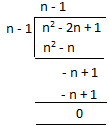n2 – 2n + 1 by n – 1 = n – 1

(ii) m2 – 2mn + n2 by m – n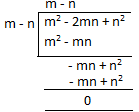m2 – 2mn + n2 by m – n = m – n

(iii) 4a2 + 4a + 1 by 2a + 1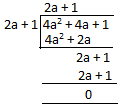4a2 + 4a + 1 by 2a + 1 = 2a + 1

(iv) p2 + 4p + 4 by p + 2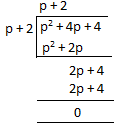p2 + 4p + 4 by p + 2 = p + 2

(v) x2 + 4xy + 4y2 by x + 2y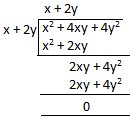x2 + 4xy + 4y2 by x + 2y = x + 2y

3. The area of a rectangle is 6x2 – 4xy – 10y2 square unit and its length is 2x + 2y unit. Find its breadth.

Solution:

It is given that

Area of a rectangle = 6x2 – 4xy – 10y2 square unit

Length = 2x + 2y unit

We know that

So we get

= (6x2 – 4xy – 10y2)/ (2x + 2y)

= 3x – 5y units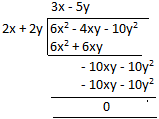4. The area of a rectangular field is 25x2 + 20xy + 3y2 square unit. If its length is 5x + 3y unit, find its breadth. Hence, find its perimeter.

Solution:

It is given that

Area of a rectangular field = 25x2 + 20xy + 3y2 square unit

Length = 5x +3y unit

We know that

So we get

= (25x2 + 20xy + 3y2)/ (5x + 3y)

= 5x + y units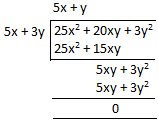Now the perimeter of the rectangular field = 2 (length + breadth)

Substituting the values

= 2 (5x + 3y + 5x + y)

So we get

= 2 (10x + 4y)

= 20x + 8y

5. Divide:

(i) 2m3n5 by – mn

(ii) 5x2 – 3x by x

(iii) 10x3y – 9xy2 – 4x2y2 by xy

(iv) 3y3 – 9ay2 – 6ab2y by – 3y

(v) x5 – 15x4 – 10x2 by – 5x2

Solution:

(i) 2m3n5 by – mn

It can be written as

= 2m3n5/ -mn

= -2m2n4

(ii) 5x2 – 3x by x

It can be written as

= (5x2 – 3x)/ x

Separating the terms

= 5x2/x – 3x/x

= 5x – 3

(iii) 10x3y – 9xy2 – 4x2y2 by xy

It can be written as

= (10x3y – 9xy2 – 4x2y2)/ xy

Separating the terms

= 10x3y/xy – 9xy2/xy – 4x2y2/xy

= 10x2 – 9y – 4xy

(iv) 3y3 – 9ay2 – 6ab2y by – 3y

It can be written as

= (3y3 – 9ay2 – 6ab2y)/ -3y

Separating the terms

= 3y3/-3y – 9ay2/-3y – 6ab2y/ -3y

= -y2 + 3ay + 2ab2

(v) x5 – 15x4 – 10x2 by – 5x2

It can be written as

= (x5 – 15x4 – 10x2)/ -5x2

Separating the terms

= x5/-5x2 – 15x4/-5x2 – 10x2/-5x2

= -1/5x3 + 3x2 + 2

#### Exercise 11E page: 133

Simplify:

1. x/2 + x/4

Solution:

x/2 + x/4

Taking LCM

= (2x + x)/4

= 3x/4

2. a/10 + 2a/5

Solution:

a/10 + 2a/5

Taking LCM

= (a + 4a)/ 10

= 5a/10

= a/2

3. y/4 + 3y/5

Solution:

y/4 + 3y/5

Taking LCM

= (5y + 12y)/ 20

= 17y/20

4. x/2 – x/8

Solution:

x/2 – x/8

Taking LCM

= (4x – x)/ 8

= 3x/8

5. 3y/4 – y/5

Solution:

3y/4 – y/5

Taking LCM

= (15y – 4y)/ 20

= 11y/20

6. 2p/3 – 3p/5

Solution:

2p/3 – 3p/5

Taking LCM

= (10p – 9p)/ 15

= p/15

7. k/2 + k/3 + 2k/5

Solution:

k/2 + k/3 + 2k/5

Here the LCM of 2, 3 and 5 is 30

= (15k + 10k + 12k)/ 30

= 37k/30

8. 2x/5 + 3x/4 – 3x/5

Solution:

2x/5 + 3x/4 – 3x/5

Here the LCM of 5 and 4 is 20

= (8x + 15x – 12x)/ 20

= 11x/20

9. 4a/7 – 2a/3 + a/7

Solution:

4a/7 – 2a/3 + a/7

Here the LCM of 3 and 7 is 21

= (12a – 14a + 3a)/ 21

= a/21

10. 2b/5 – 7b/15 + 13b/3

Solution:

2b/5 – 7b/15 + 13b/3

Here the LCM of 3, 5 and 15 is 15

= (6b – 7b + 65b)/ 15

= 64b/15

11. 6k/7 – (8k/9 – k/3)

Solution:

6k/7 – (8k/9 – k/3)

Here the LCM of 7, 9 and 3 is 63

= [54k – (56k – 21k)]/ 63

By further calculation

= (54k – 35k)/ 63

= 19k/63

12. 3a/8 + 4a/5 – (a/2 + 2a/5)

Solution:

3a/8 + 4a/5 – (a/2 + 2a/5)

Here the LCM of 8, 5 and 2 is 40

= [15a + 32a – (20a + 16a)]/ 40

By further calculation

= (47a – 36a)/ 40

= 11a/40

13. x + x/2 + x/3

Solution:

x + x/2 + x/3

Taking LCM

= (6x + 3x + 2x)/ 6

= 11x/6

14. y/5 + y – 19y/15

Solution:

y/5 + y – 19y/15

Here the LCM of 5 and 15 is 15

= (3y + 15y – 19y)/ 15

So we get

= – y/15

15. x/5 + (x + 1)/2

Solution:

x/5 + (x + 1)/2

Here the LCM of 5 and 2 is 10

= (2x + 5x + 5)/ 10

= (7x + 5)/ 10

#### Exercise 11F page: 136

Enclose the given terms in brackets as required:

1. x – y – z = x – (…………)

2. x2 – xy2 – 2xy – y2 = x2 – (………….)

3. 4a – 9 + 2b – 6 = 4a – (………..)

4. x2 – y2 + z2 + 3x – 2y = x2 – (…………)

5. -2a2 + 4ab – 6a2b2 + 8ab2 = -2a (…………….)

Solution:

1. x – y – z = x – (y + z)

2. x2 – xy2 – 2xy – y2 = x2 – (xy2 + 2xy + y2)

3. 4a – 9 + 2b – 6 = 4a – (9 – 2b + 6)

4. x2 – y2 + z2 + 3x – 2y = x2 – (y2 – z2 – 3x + 2y)

5. -2a2 + 4ab – 6a2b2 + 8ab2 = -2a (a – 2b + 3ab2 – 4b2)

Simplify:

6. 2x – (x + 2y – z)

Solution:

2x – (x + 2y – z)

We can write it as

= 2x – x – 2y + z

So we get

= x – 2y + z

7. p + q – (p – q) + (2p – 3q)

Solution:

p + q – (p – q) + (2p – 3q)

We can write it as

= p + q – p + q + 2p – 3q

So we get

= 2p – q

8. 9x – (-4x + 5)

Solution:

9x – (- 4x + 5)

We can write it as

= 9x + 4x – 5

So we get

= 13x – 5

9. 6a – (- 5a – 8b) + (3a + b)

Solution:

6a – (- 5a – 8b) + (3a + b)

We can write it as

= 6a + 5a + 8b + 3a + b

So we get

= 6a + 5a + 3a + 8b + b

= 14a + 9b

10. (p – 2q) – (3q – r)

Solution:

(p – 2q) – (3q – r)

We can write it as

= p – 2q – 3q + r

So we get

= p – 5q + r

11. 9a (2b – 3a + 7c)

Solution:

9a (2b – 3a + 7c)

We can write it as

= 18ab – 27a2 + 63ca

12. – 5m (- 2m + 3n – 7p)

Solution:

– 5m (- 2m + 3n – 7p)

We can write it as

= 10m2 – 15mn + 35mp

13. – 2x (x + y) + x2

Solution:

– 2x (x + y) + x2

We can write it as

= -2x2 – 2xy + x2

So we get

= – x2 – 2xy

14. b (2b – 1/b) – 2b (b – 1/b)

Solution:

b (2b – 1/b) – 2b (b – 1/b)

We can write it as

= 2b2 – 1 – 2b2 + 2

So we get

= 1

15. 8 (2a + 3b – c) – 10 (a + 2b + 3c)

Solution:

8 (2a + 3b – c) – 10 (a + 2b + 3c)

We can write it as

= 16a + 24b – 8c – 10a – 20b – 30c

So we get

= 6a + 4b – 38c